# ICSE Class 10 Maths Question Paper Solution 2017

Solving ICSE Class 10 Maths Question Paper 2017 will give a good practice to students before the board exam. By practising the questions asked in previous year papers, students will get to know the types of questions asked in the exam. Also, they get to know the important topics from which maximum questions are asked in the exam. We have provided the answers of the 2017 Maths paper in the form of ICSE Class 10 Maths Question Paper 2017 Solutions PDF. The solutions contain the answers in steps along with the marking scheme.

The ICSE Class 10 Maths 2017 exam was conducted on 17th April 2017. The exam started at 11 am and students were allotted 2 hours 30 minutes of time duration to finish the paper. Students can download the ICSE Class 10 Maths Question Paper Solution 2017 PDF from the link below.

Students can have a look at the ICSE Class 10 Maths Question Paper Solution 2017 below: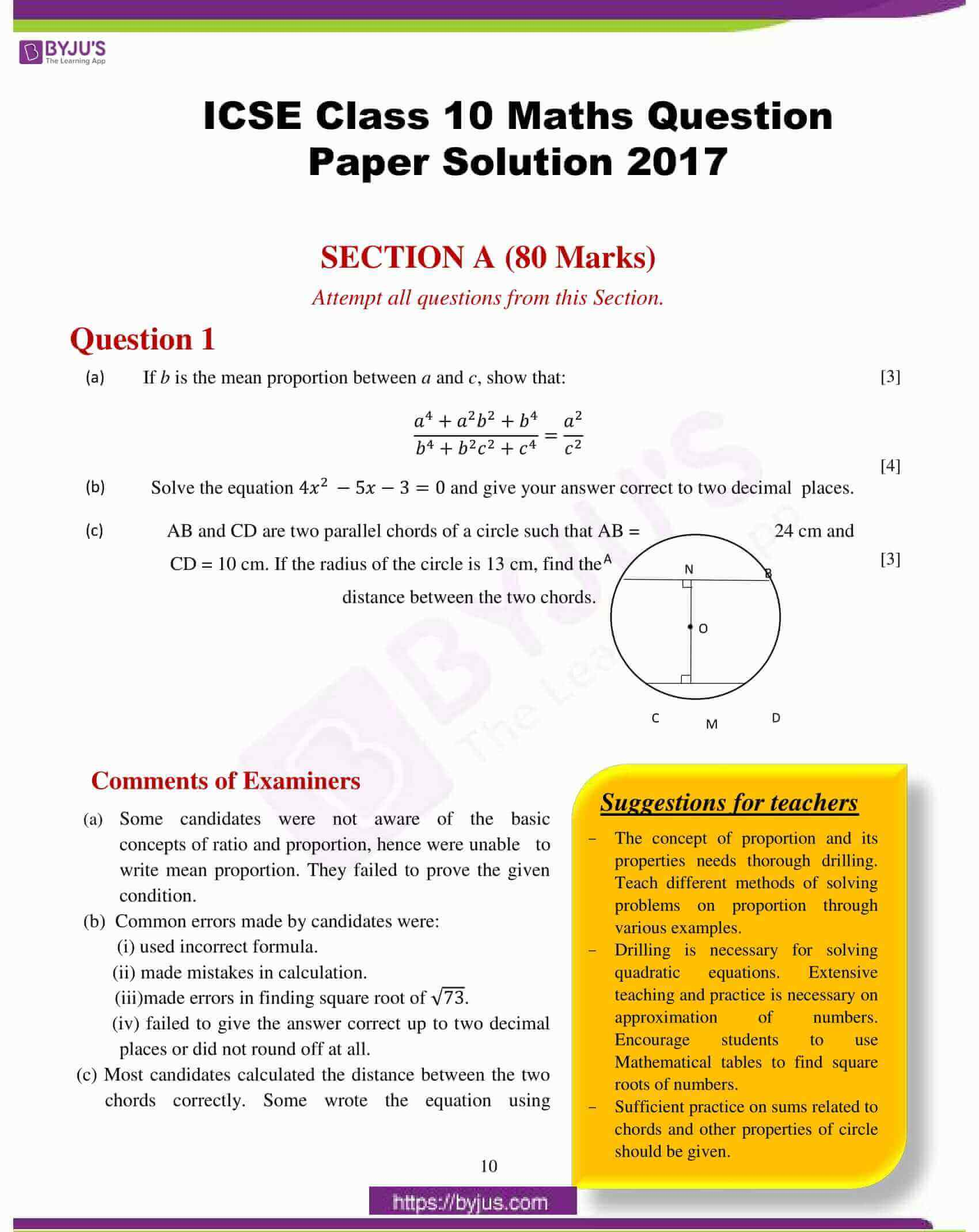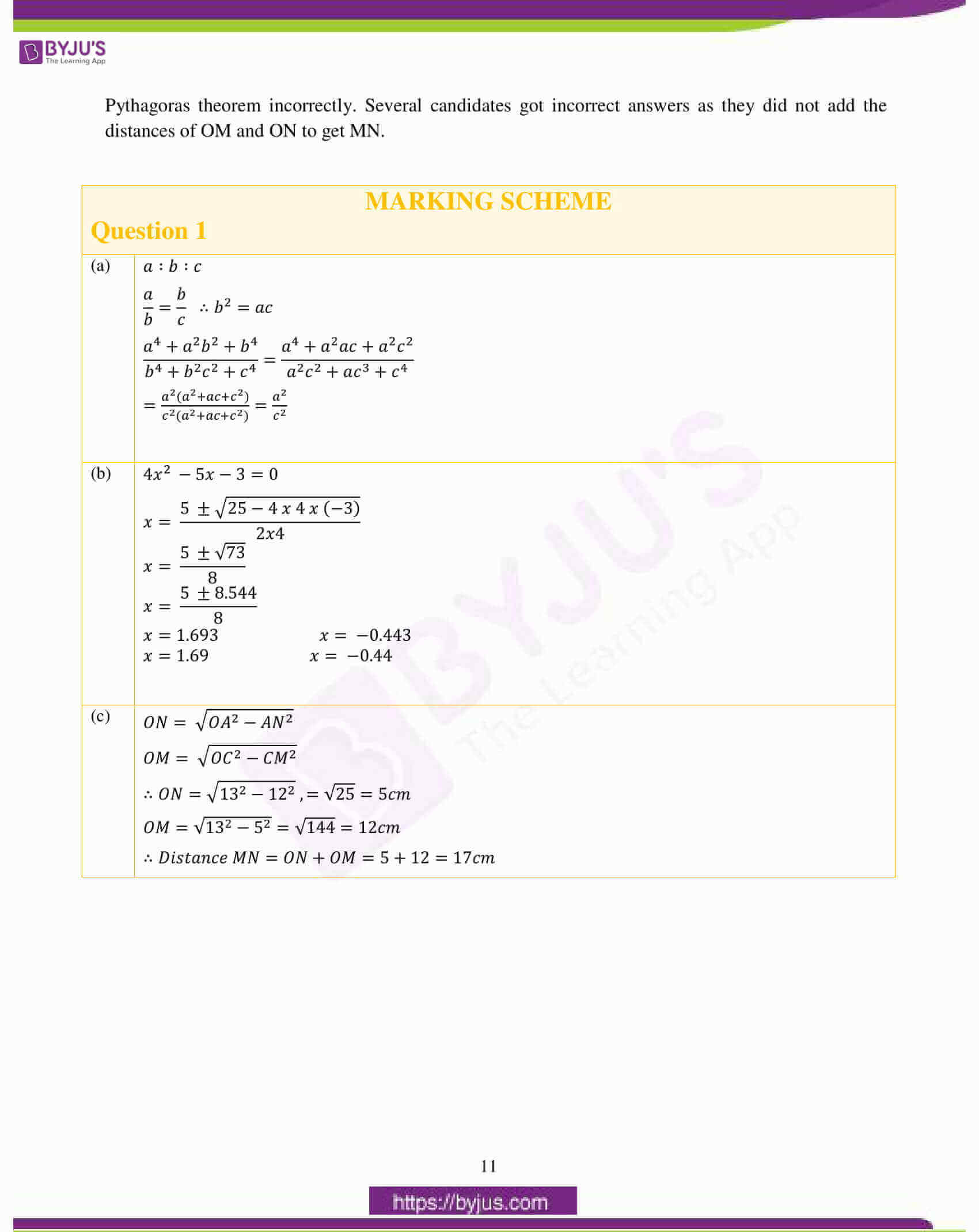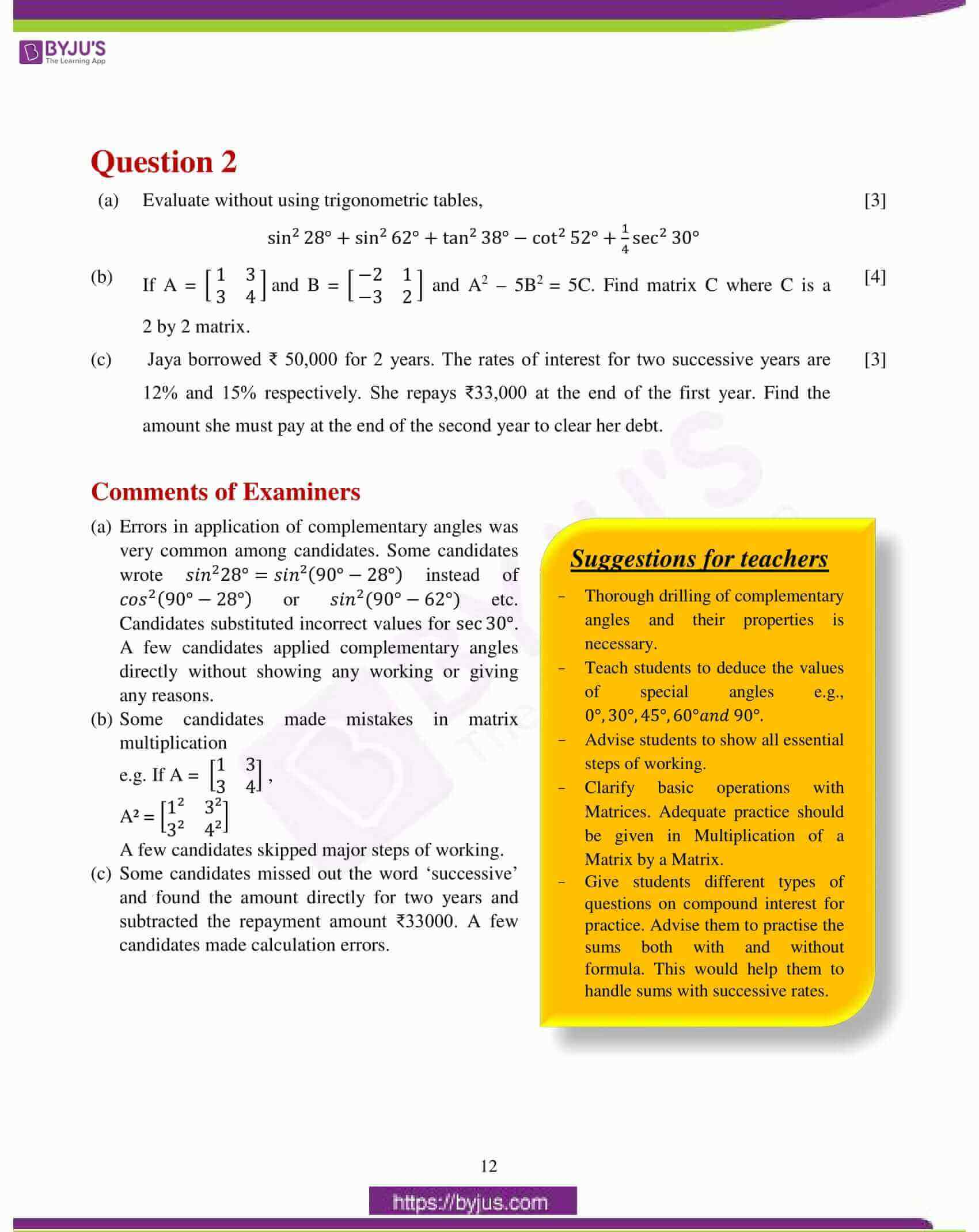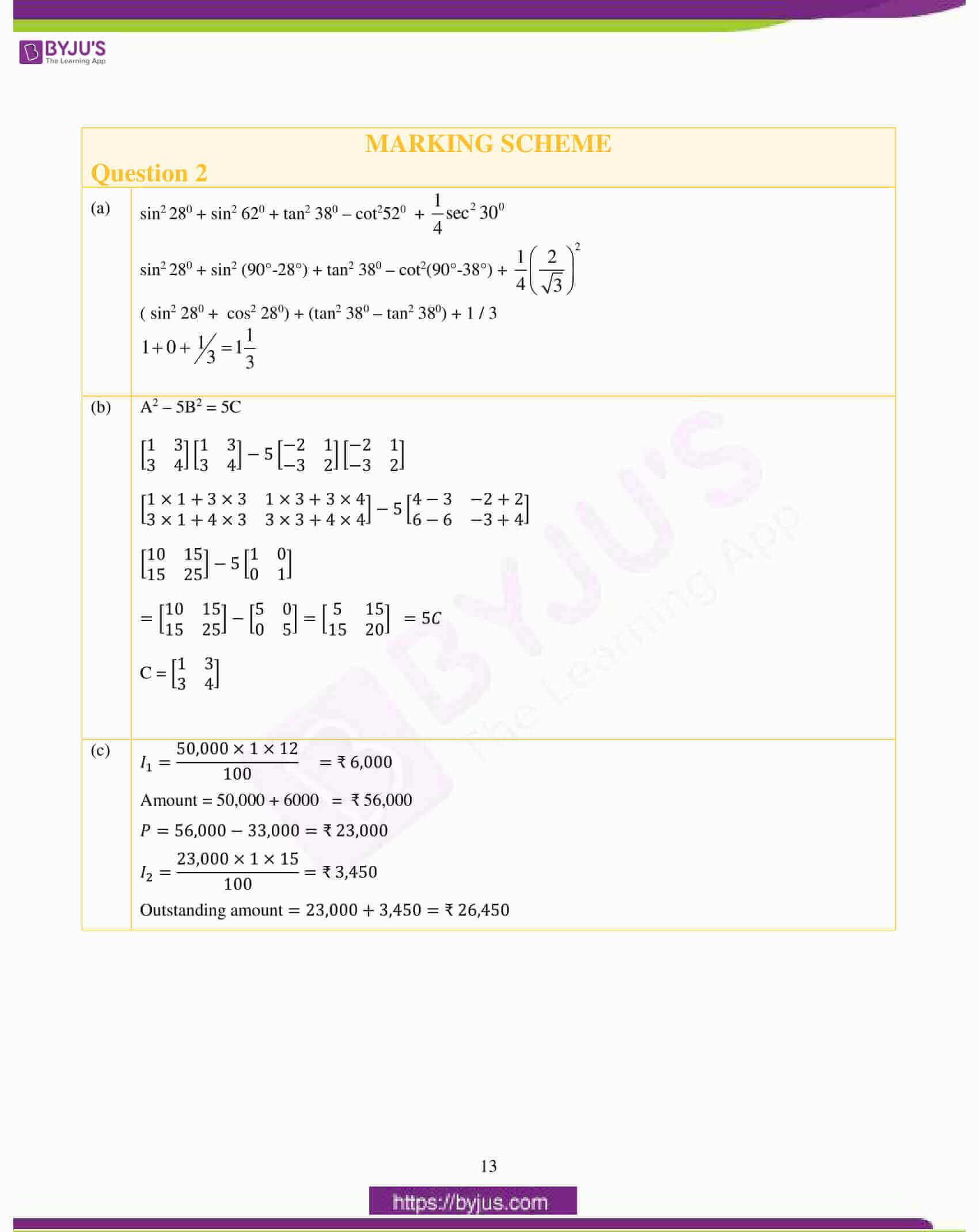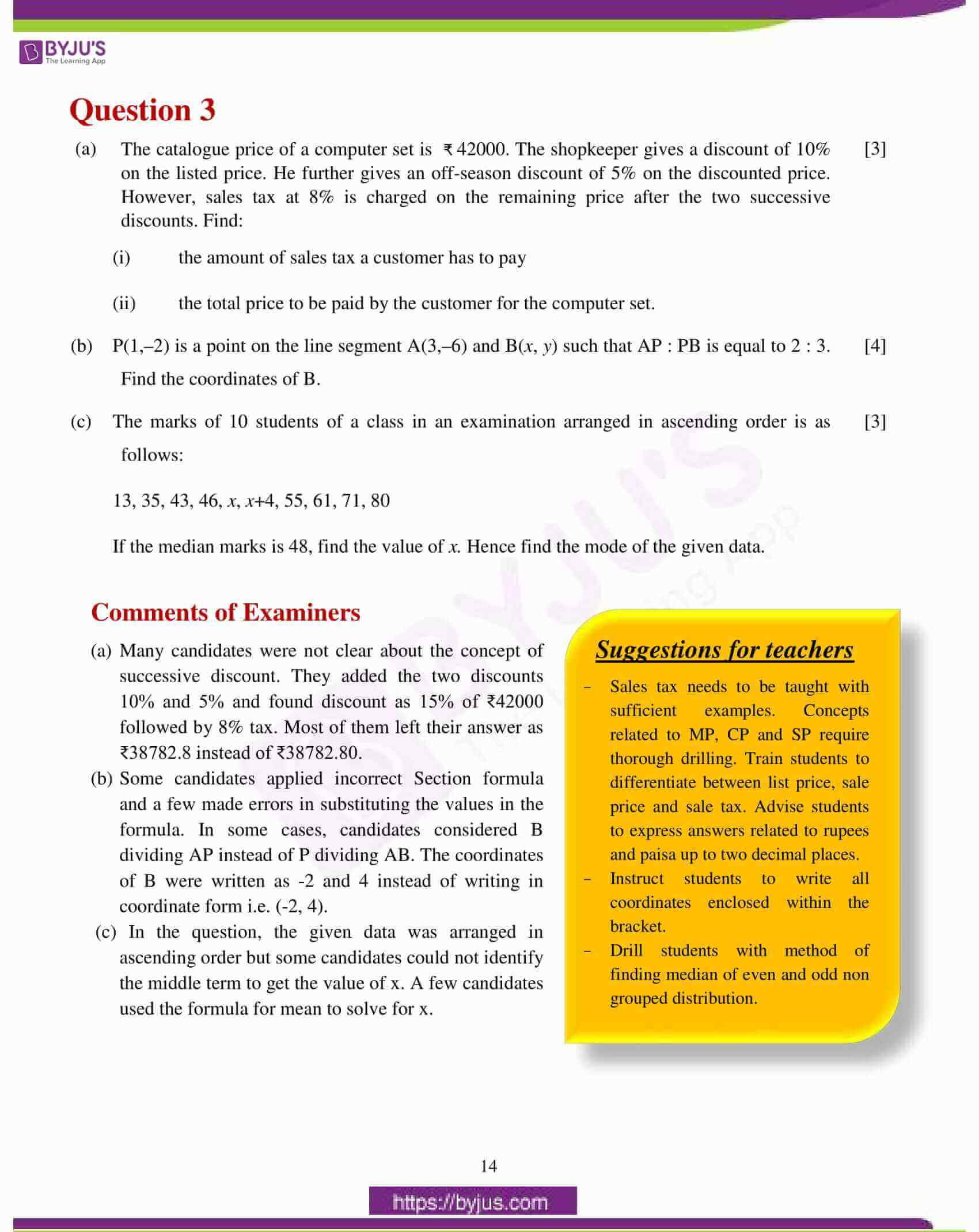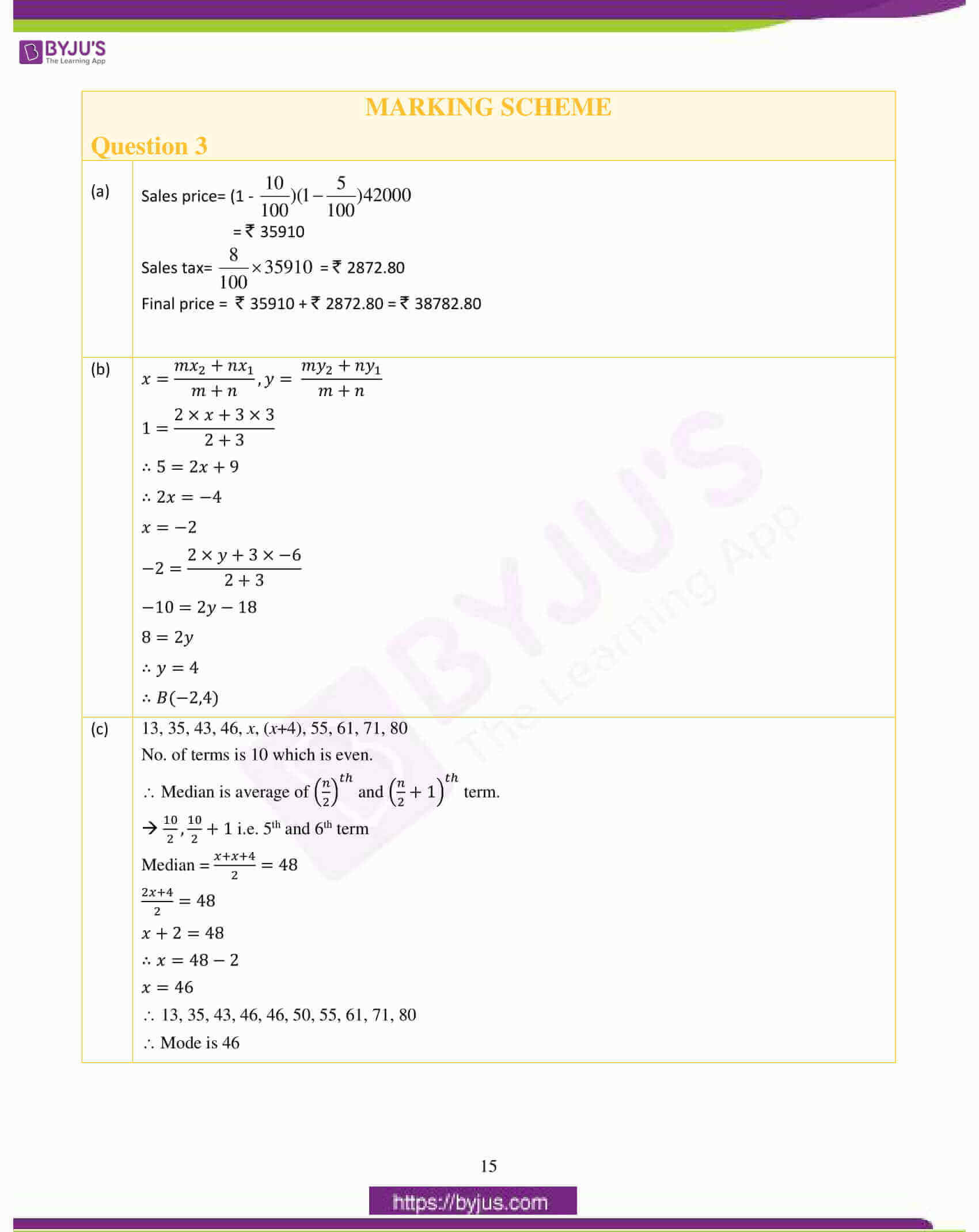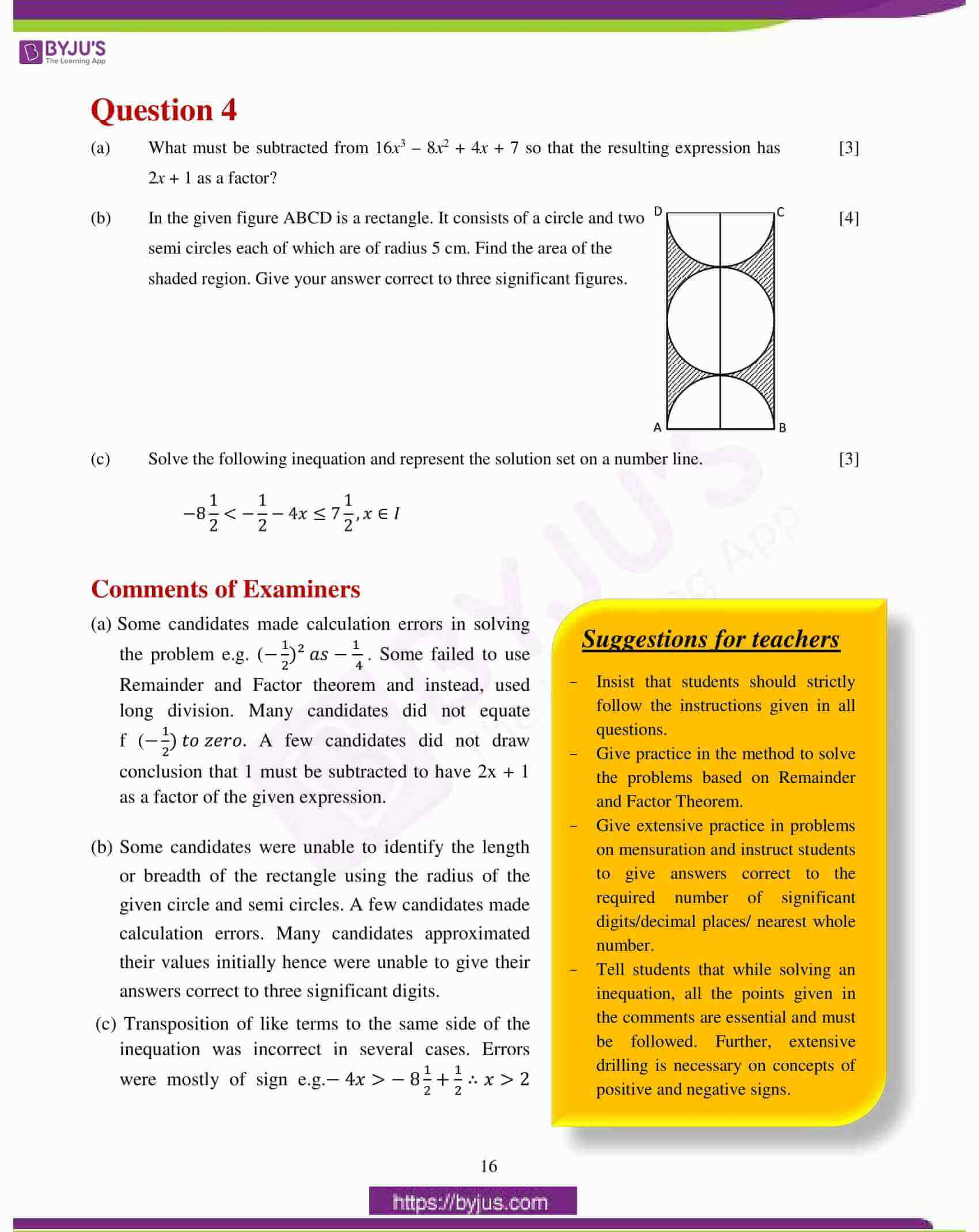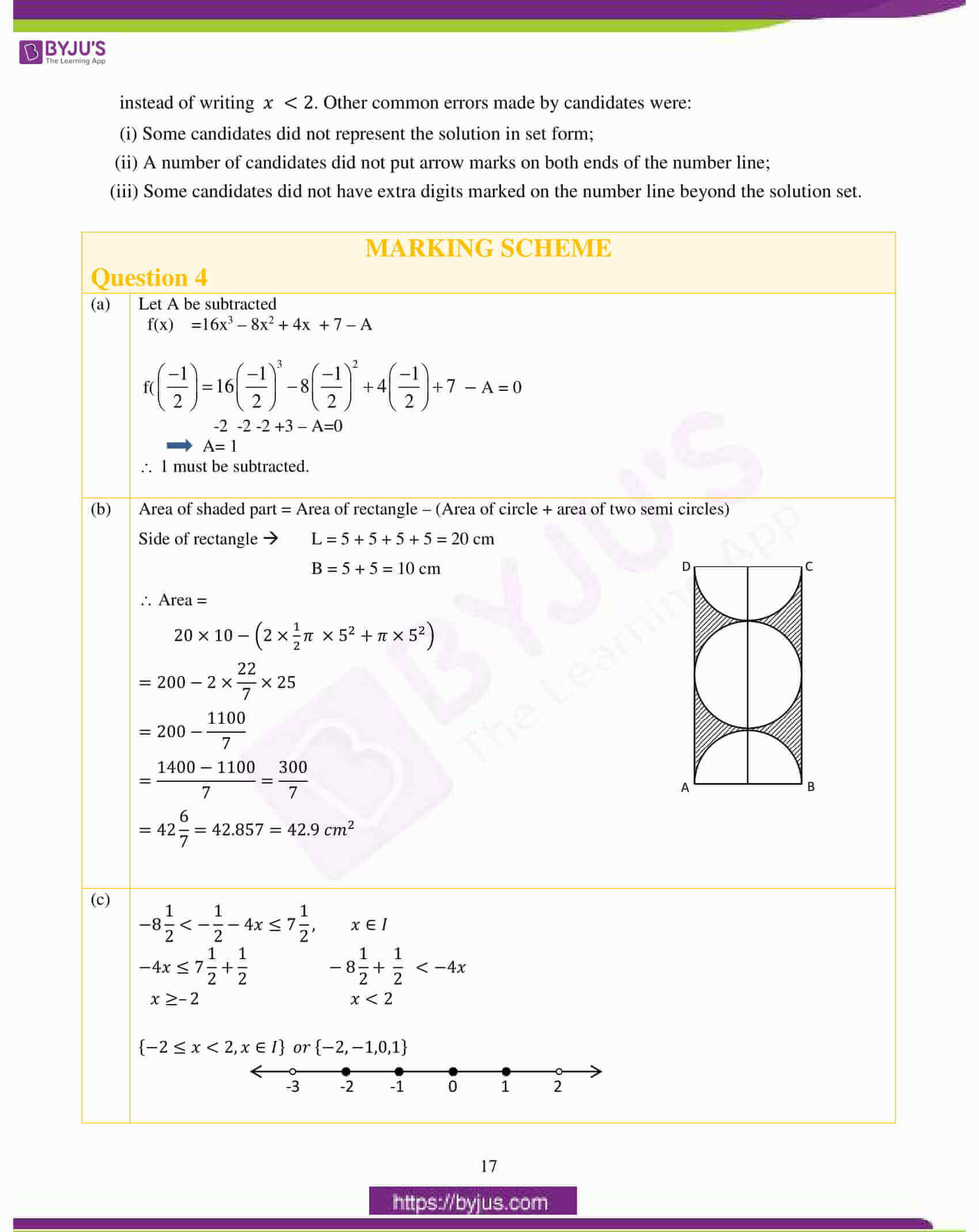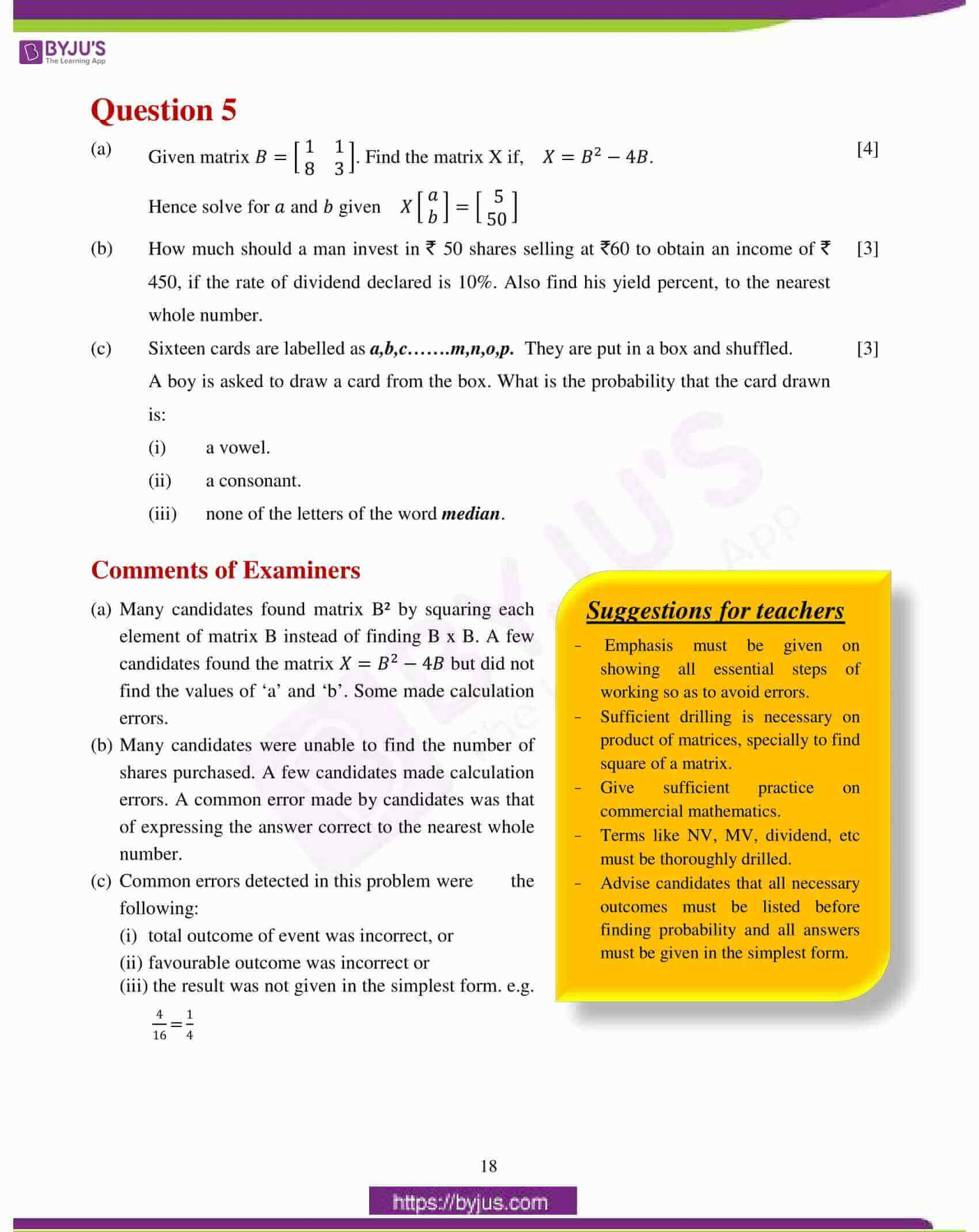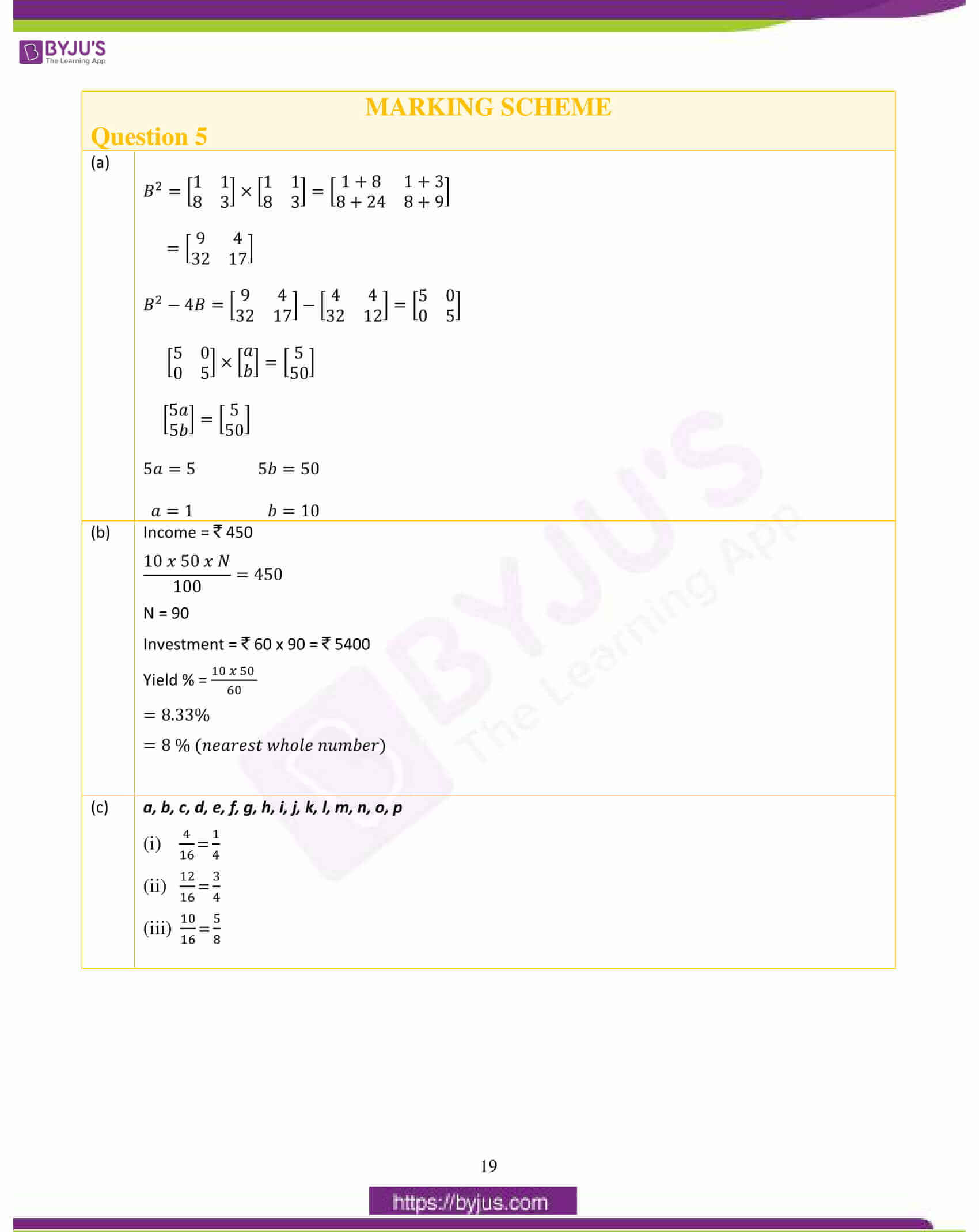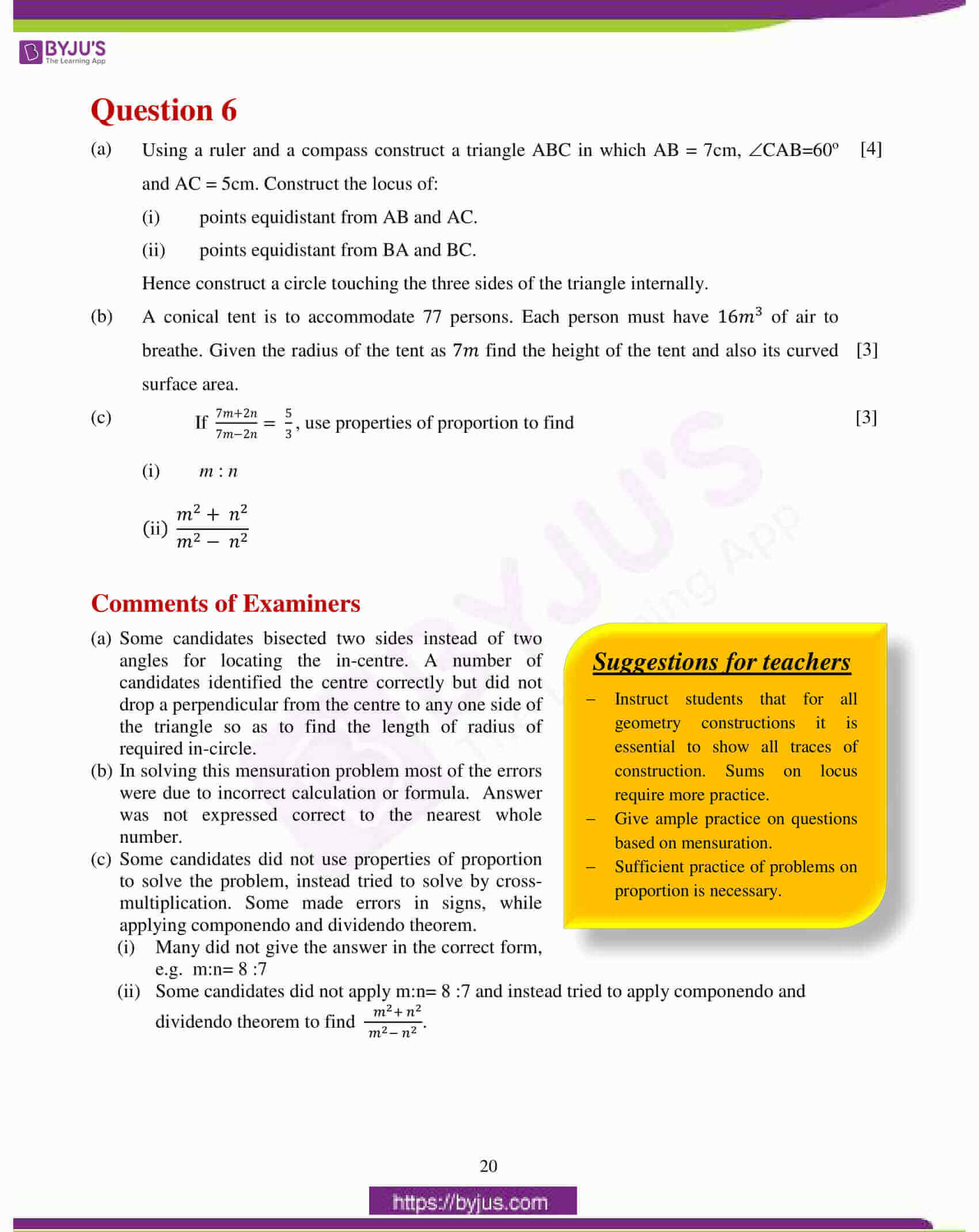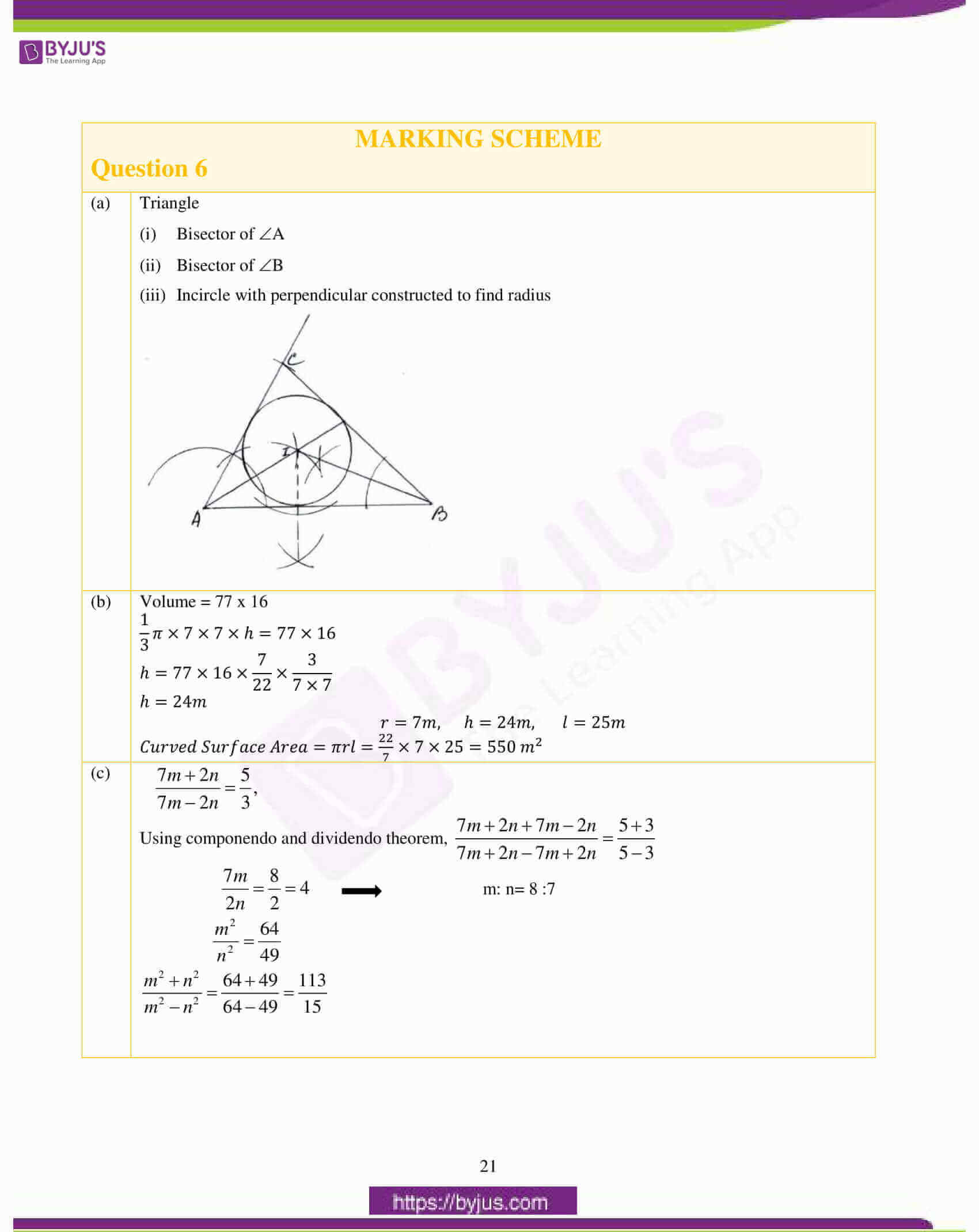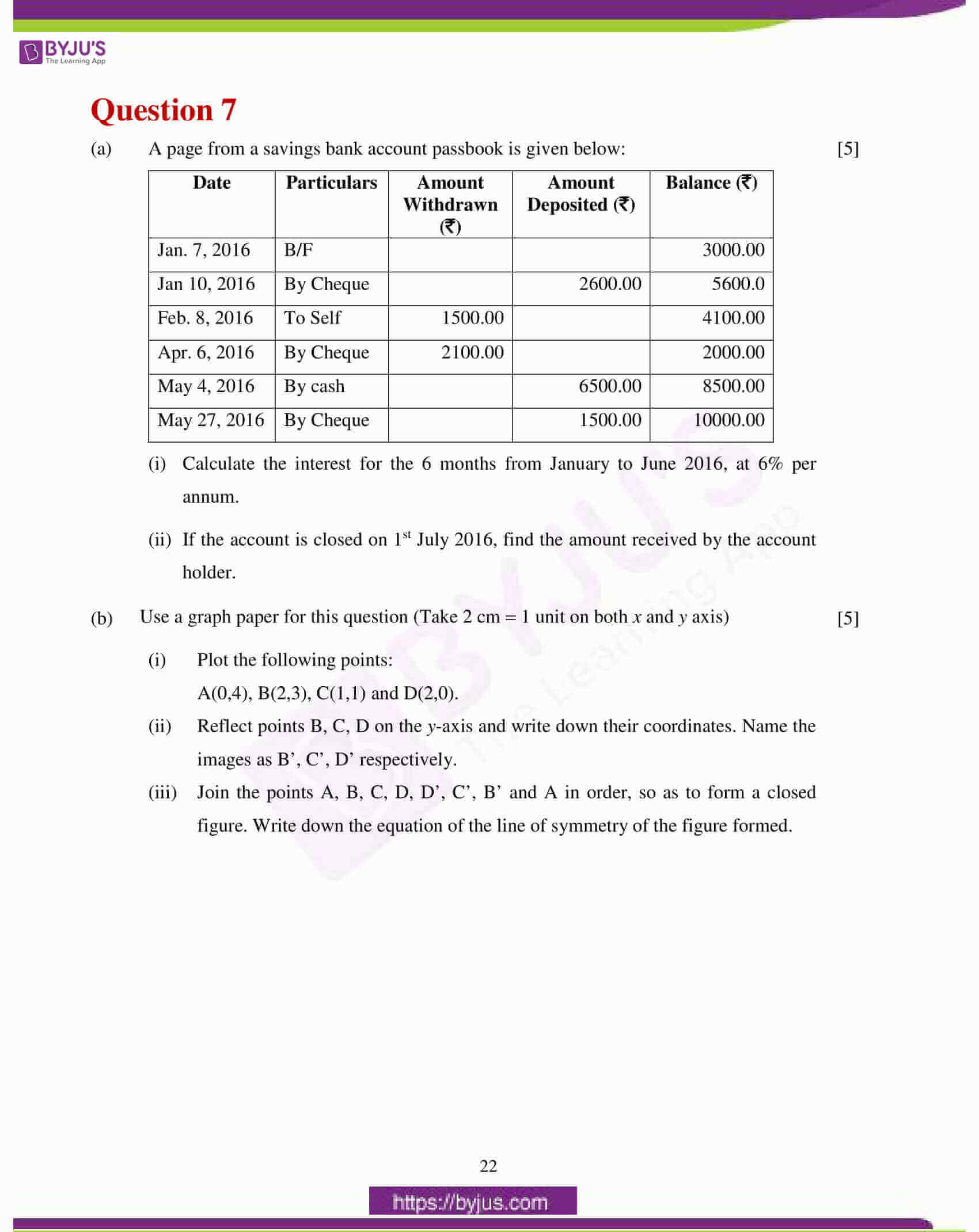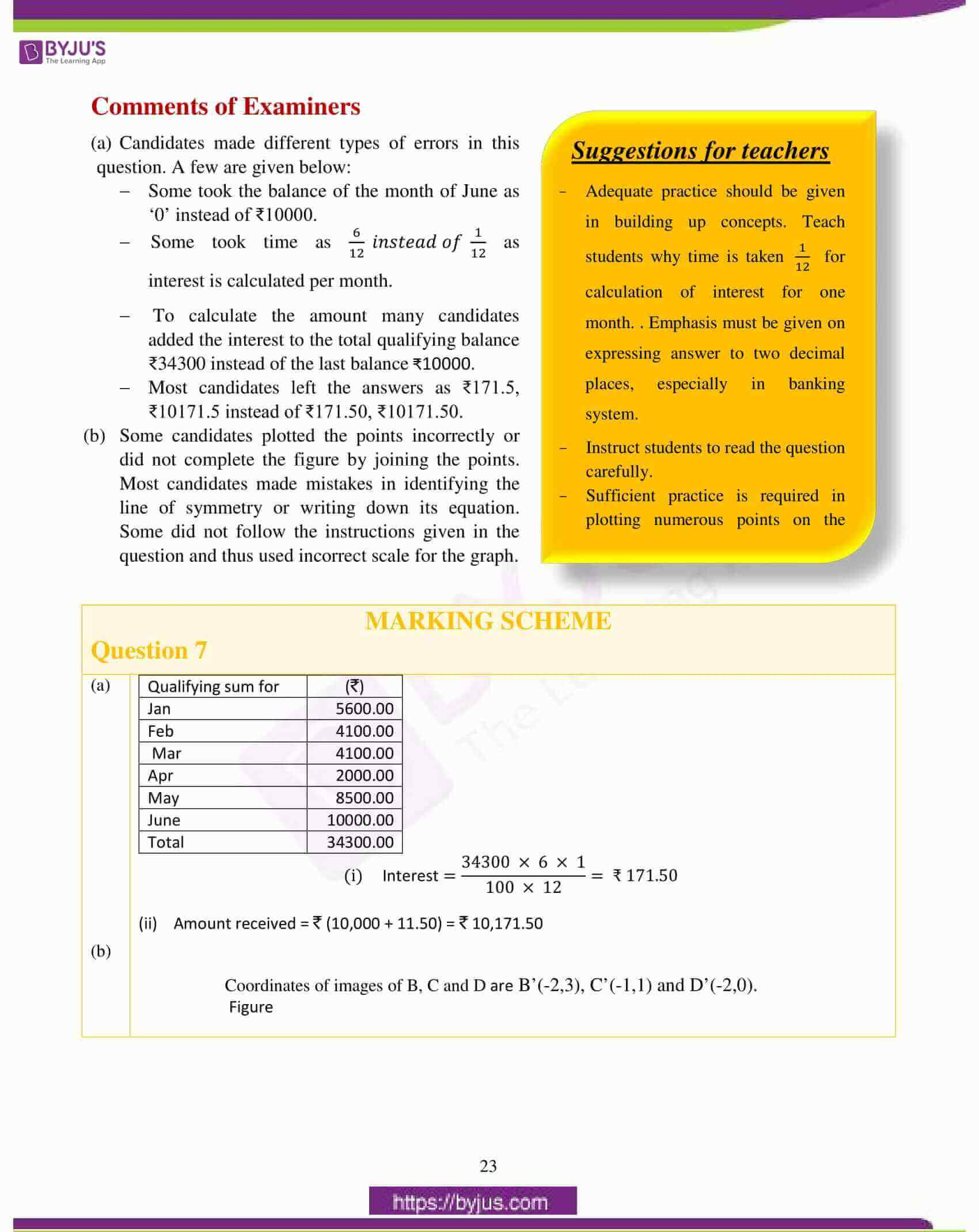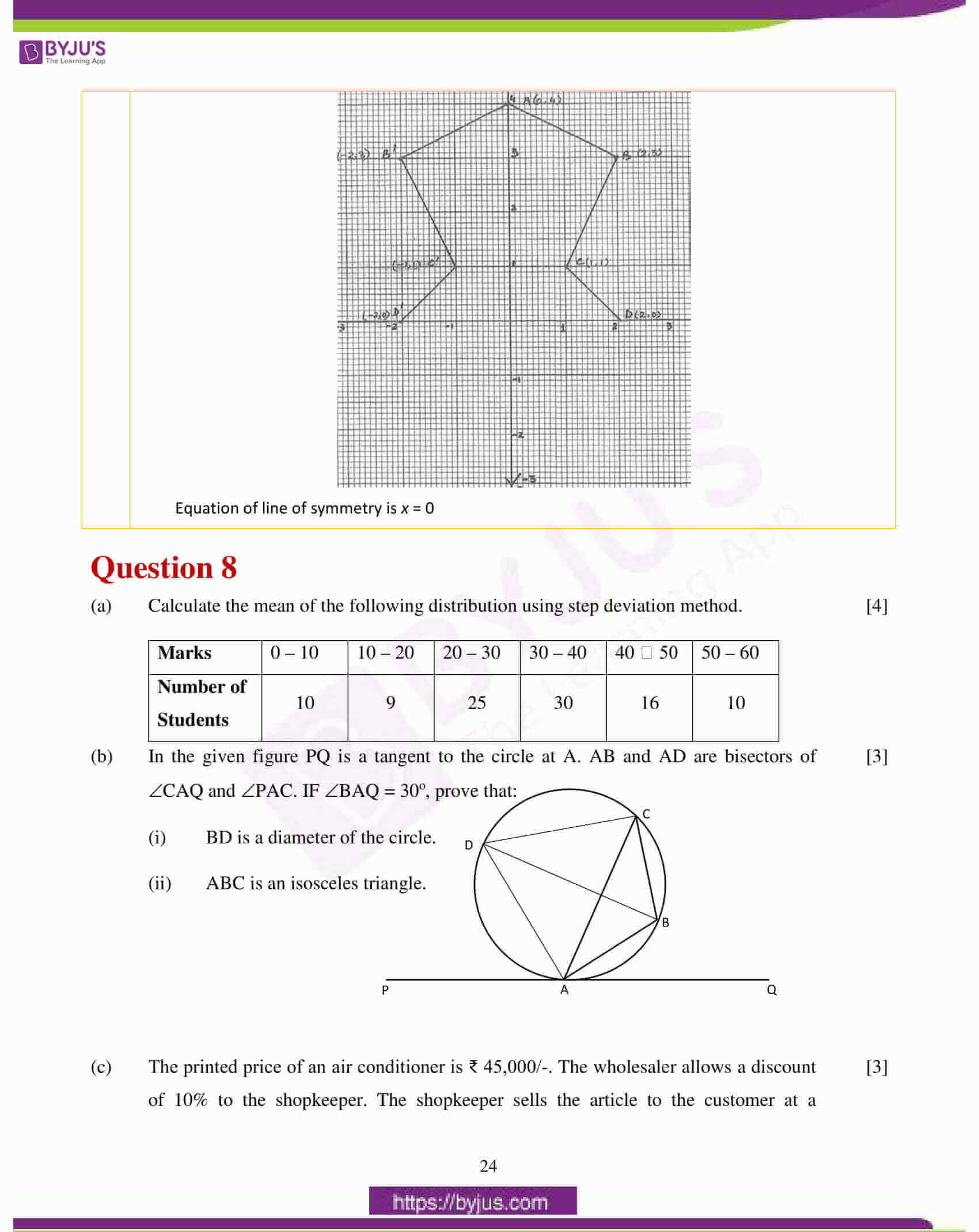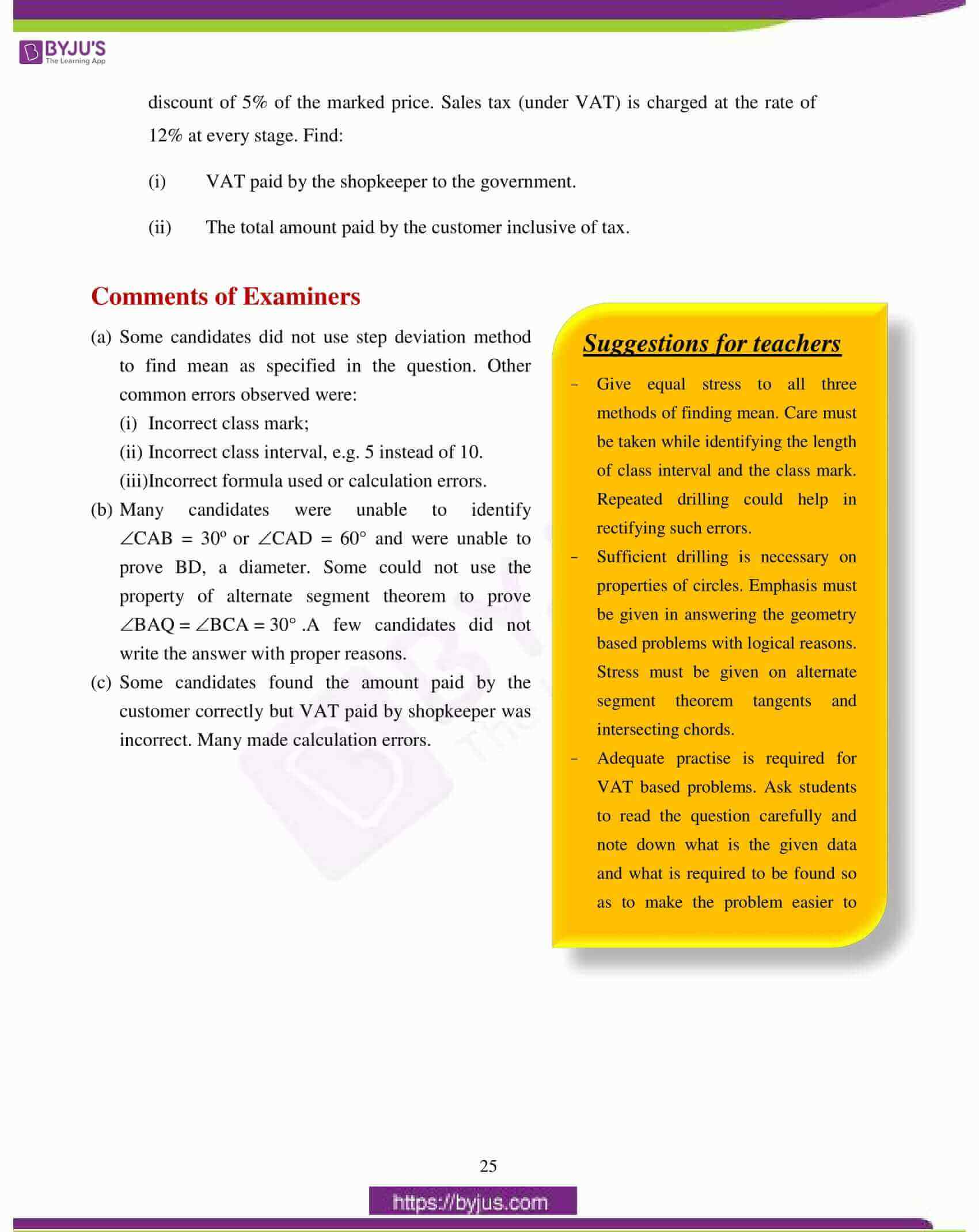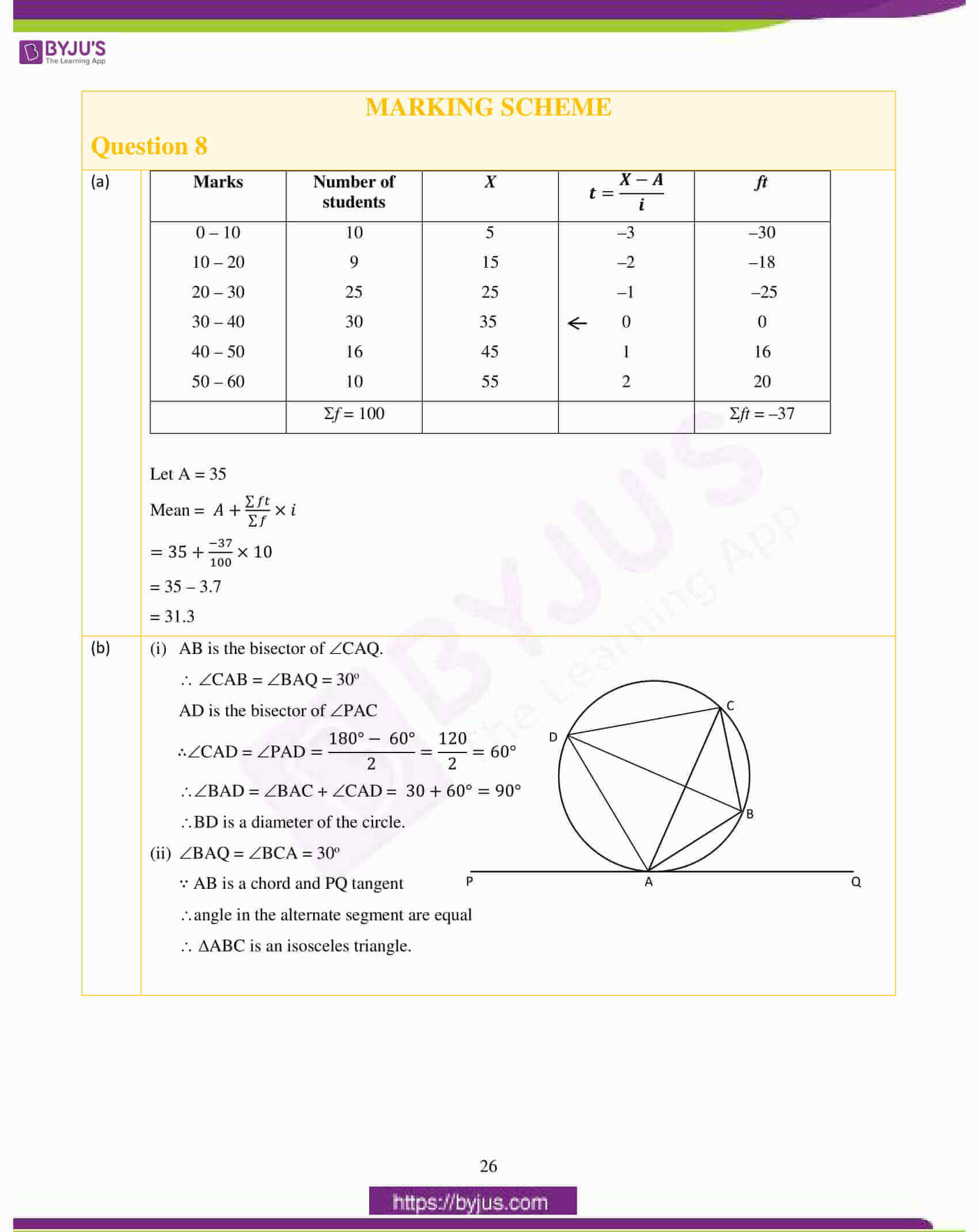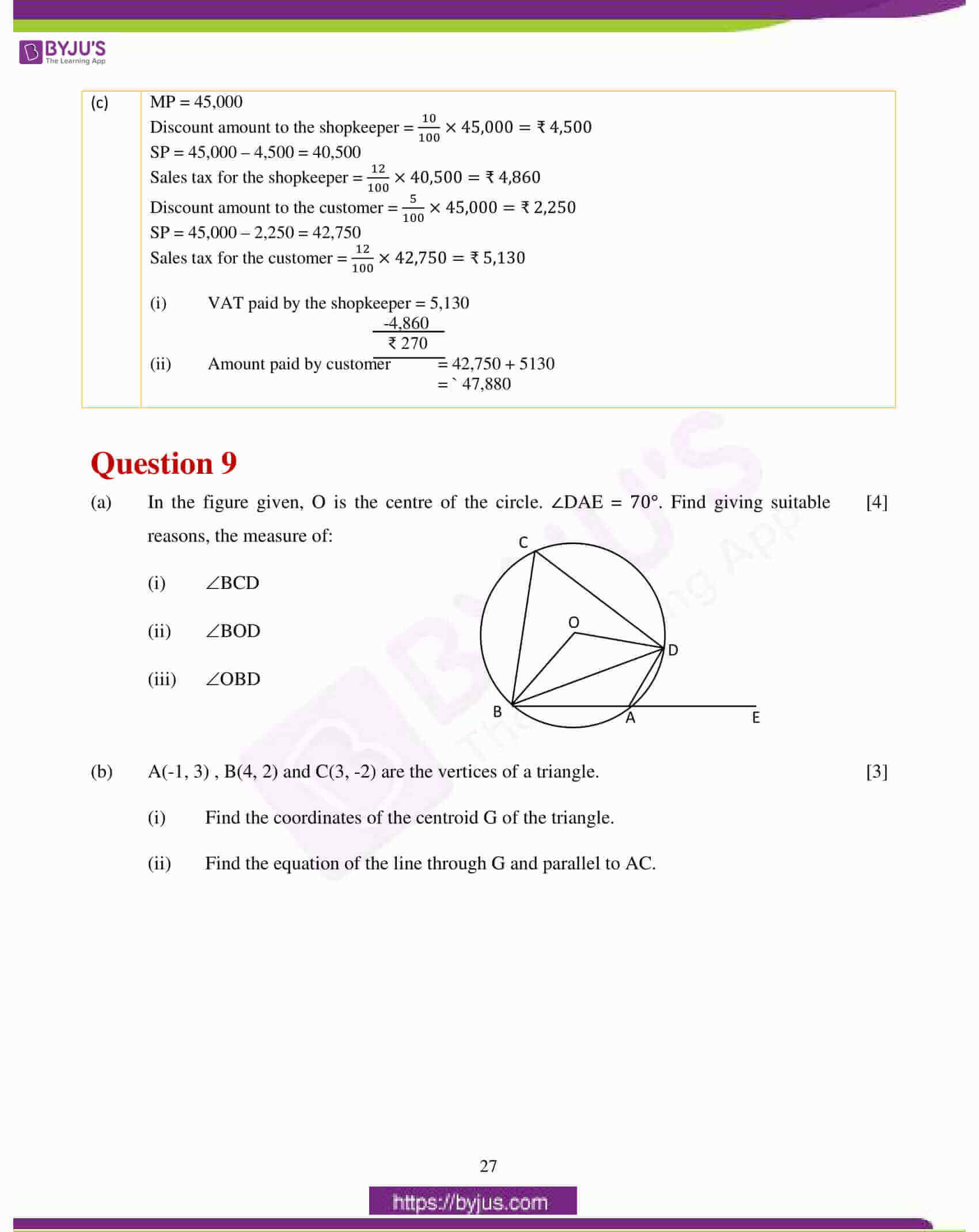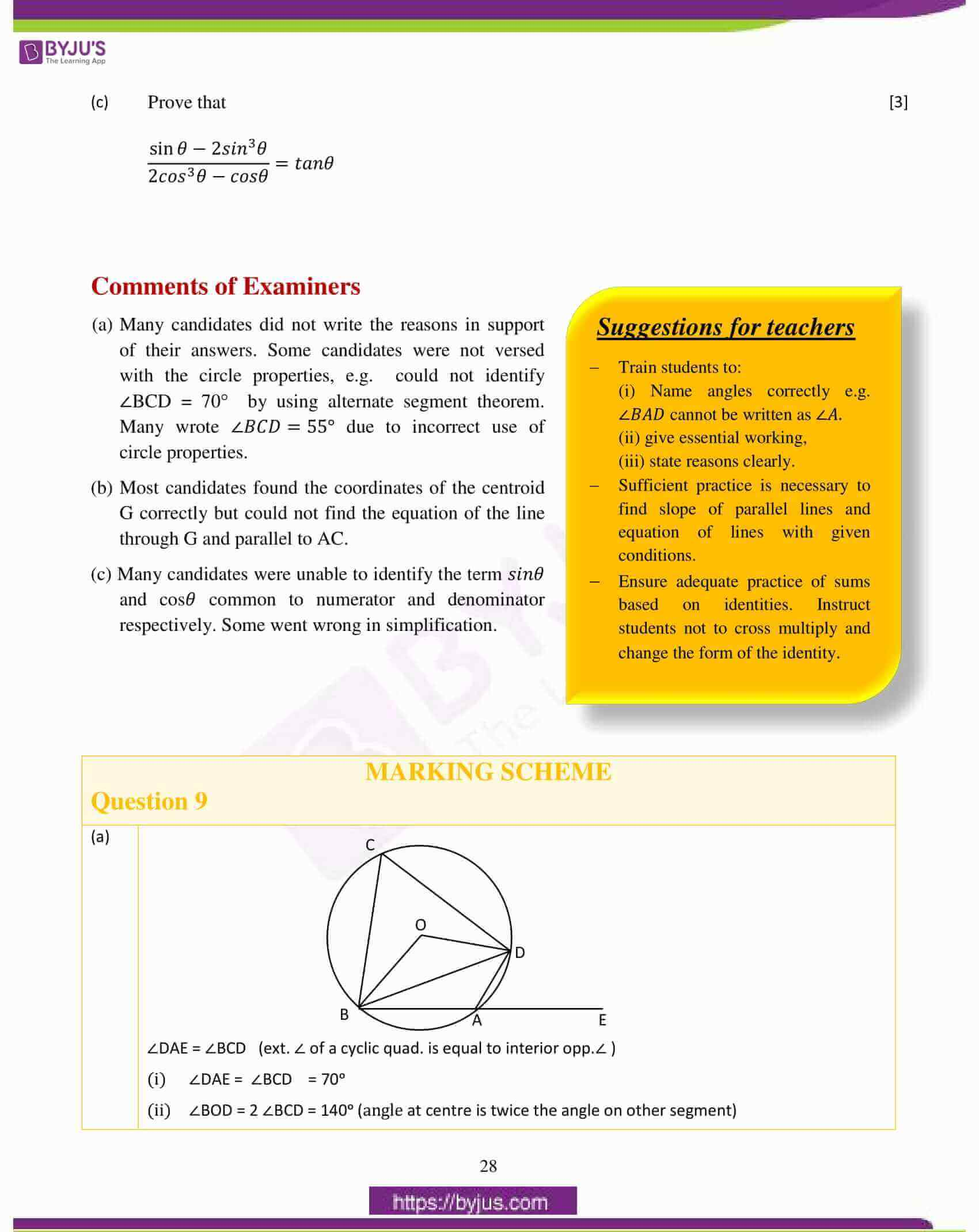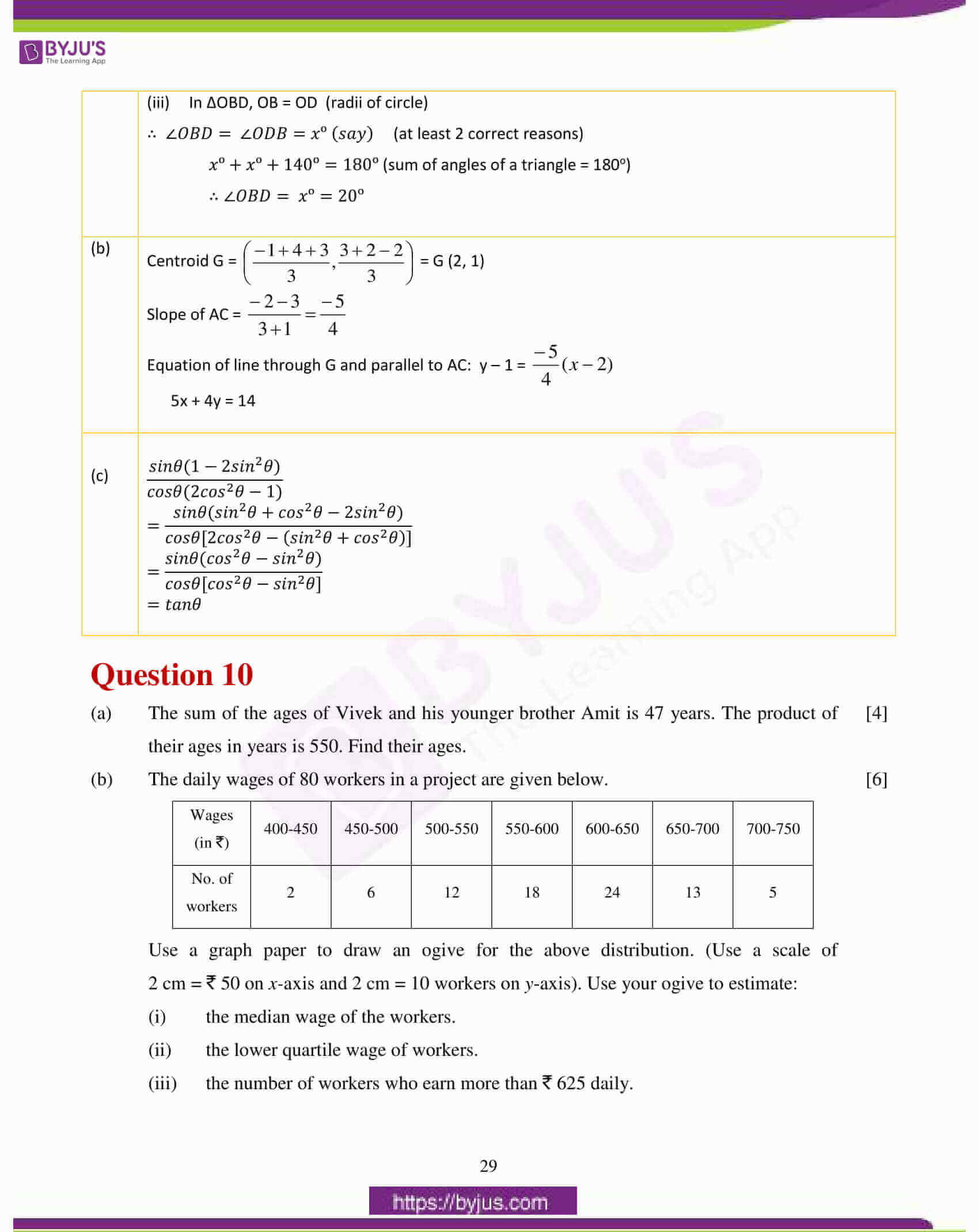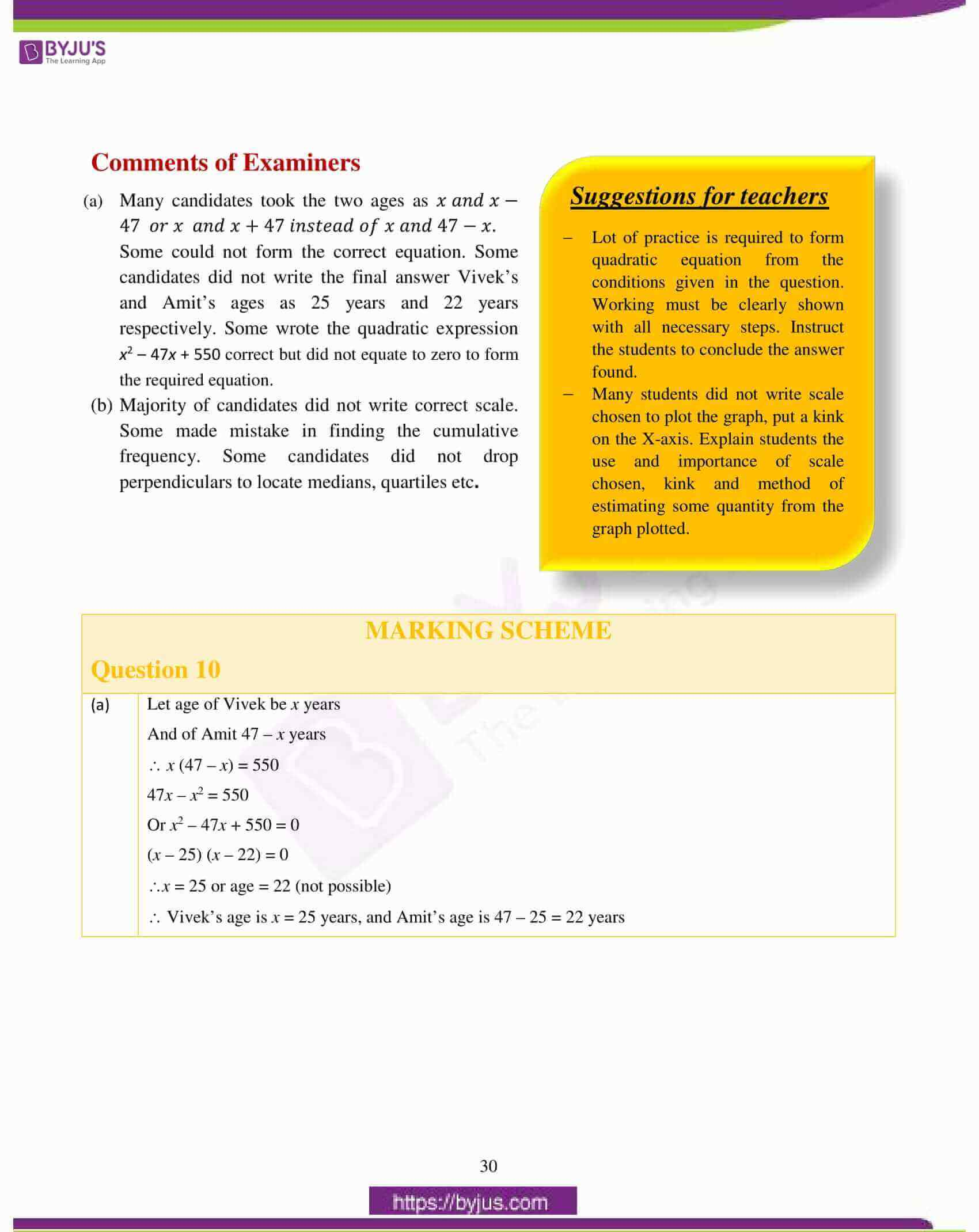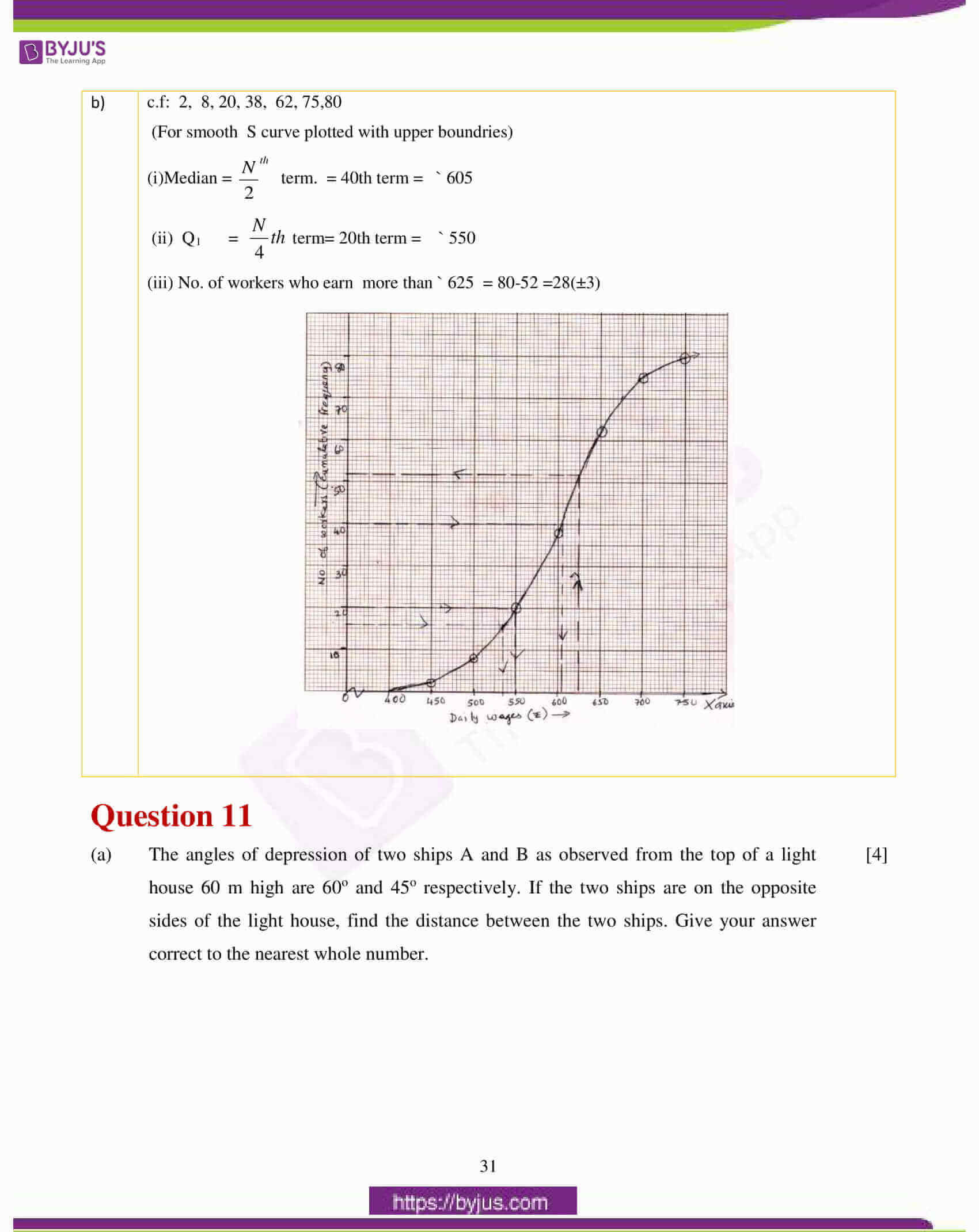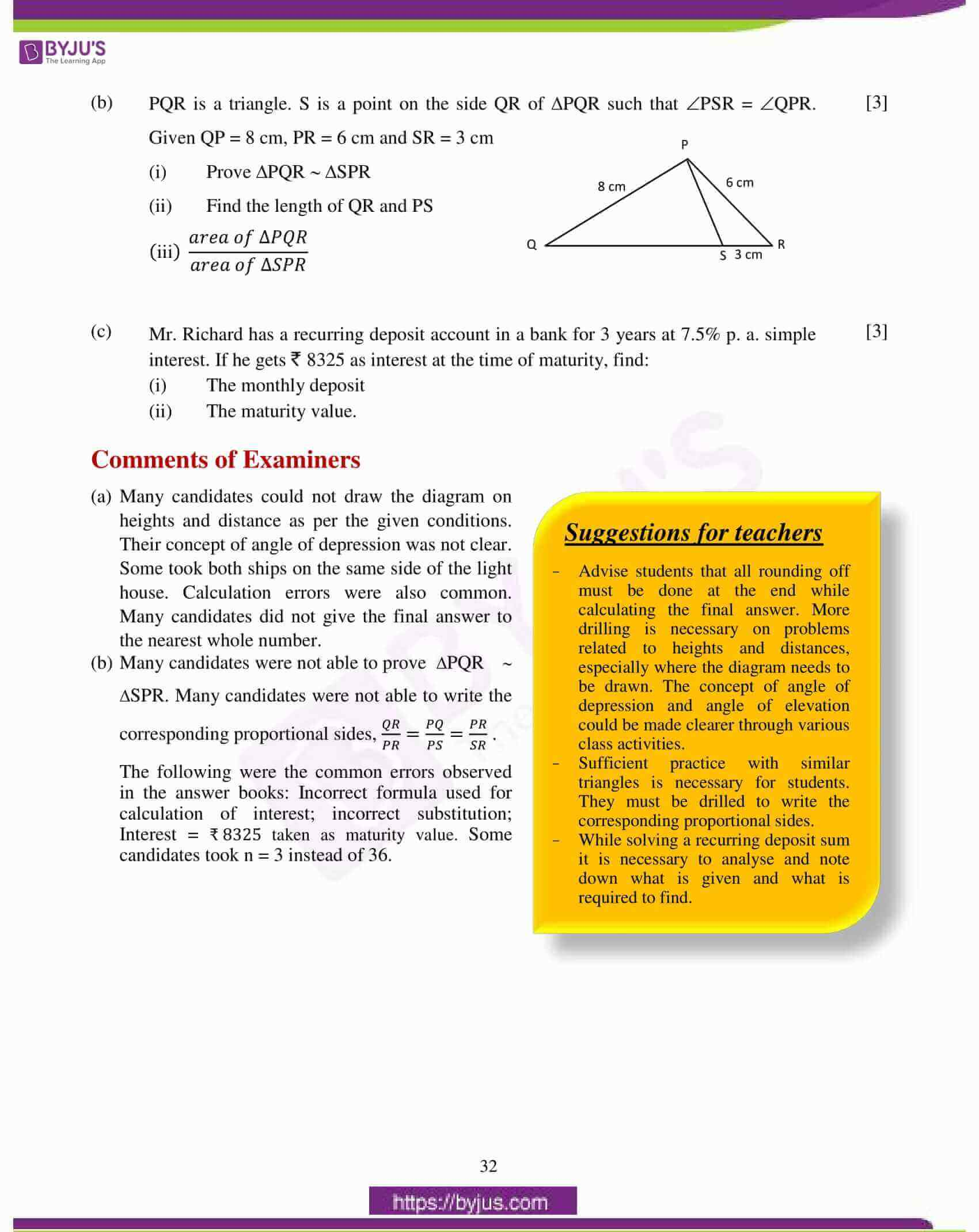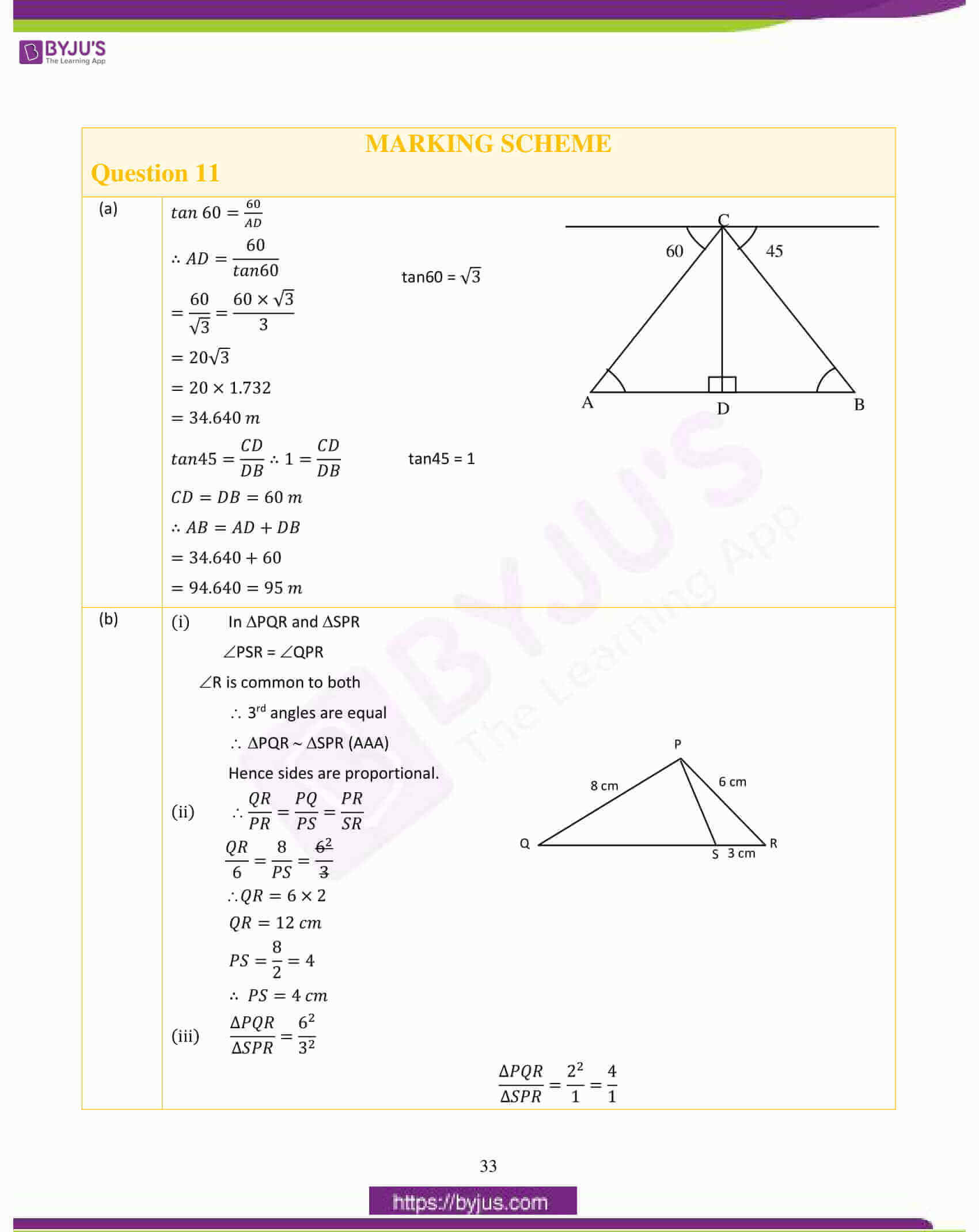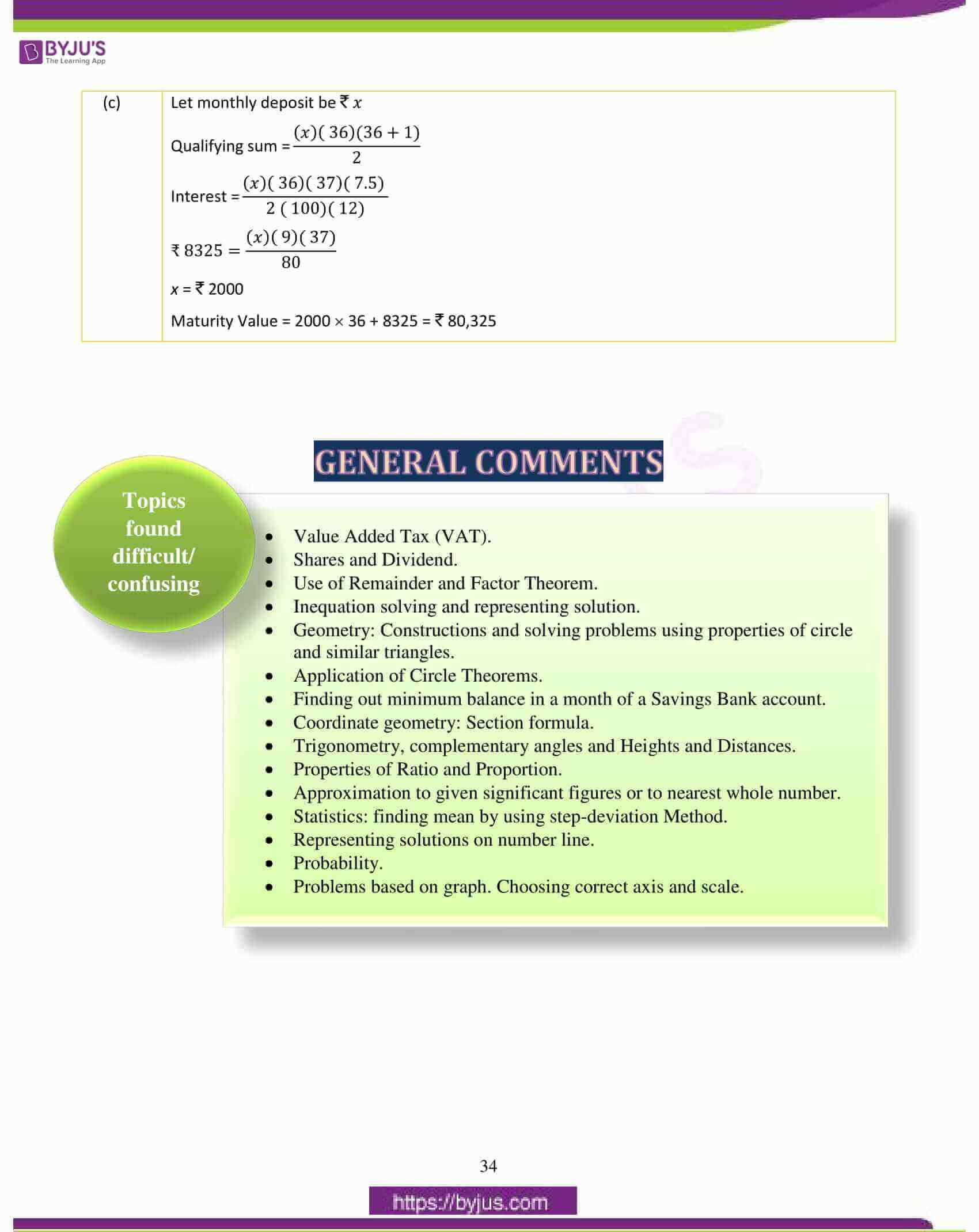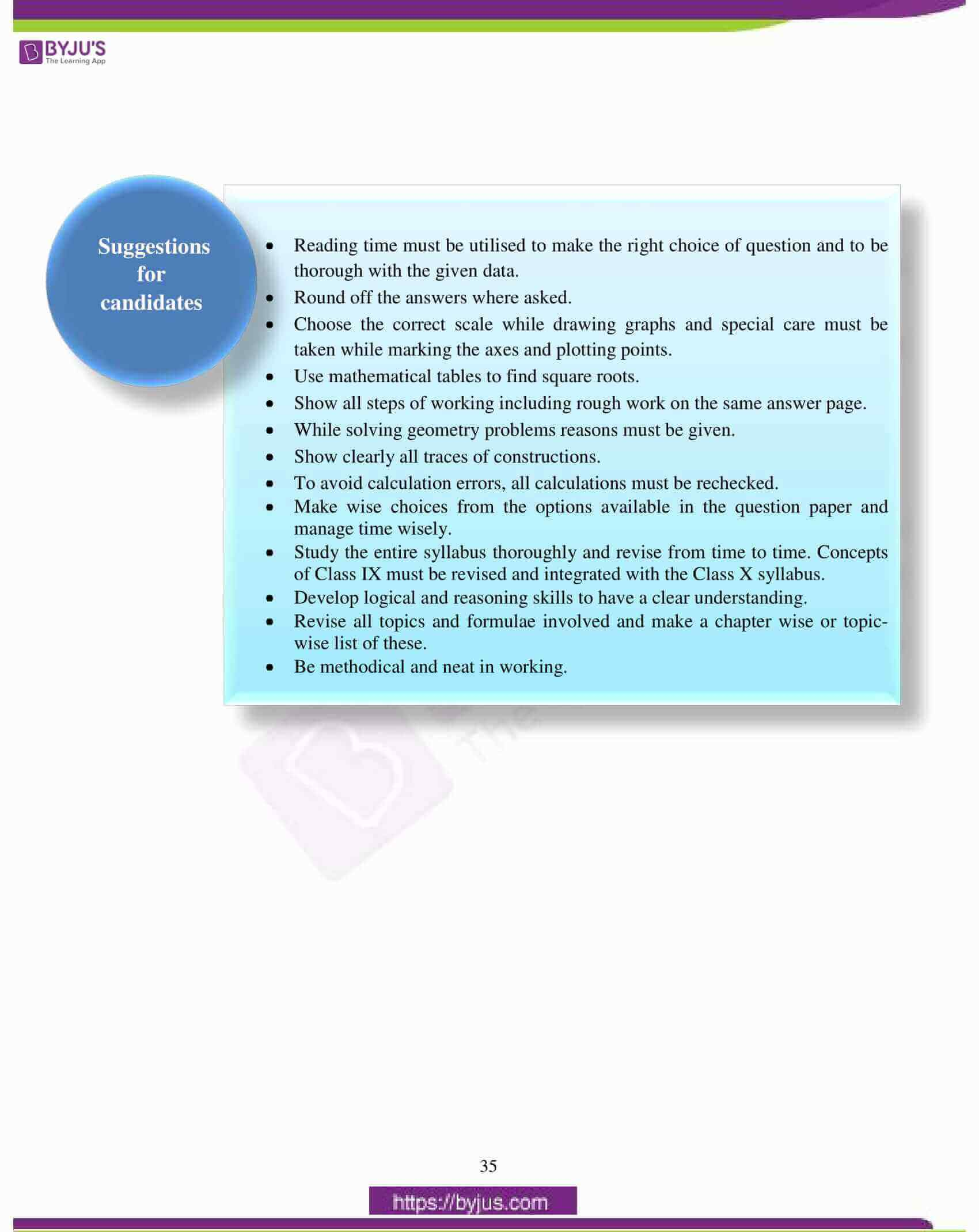## ICSE Class 10 Maths Question Paper 2017 With Solution

### Question 1:

(a) If b is the mean proportion between a and c, show that:

$$\begin{array}{l}\frac{a^{4}+a^{2}b^{2}+b^{4}}{b^{4}+b^{2}c^{2}+c^{4}} = \frac{a^{2}}{c^{2}}\end{array}$$

(b) Solve the equation 4𝑥2 − 5𝑥 − 3 = 0 and give your answer correct to two decimal places.

(c) AB and CD are two parallel chords of a circle such that AB = 24 cm and CD = 10 cm. If the radius of the circle is 13 cm, find the distance between the two chords.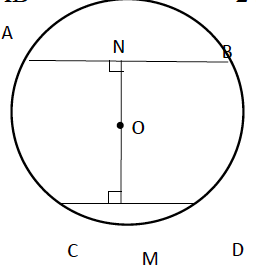(a)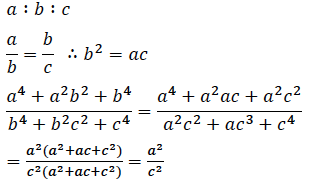(b)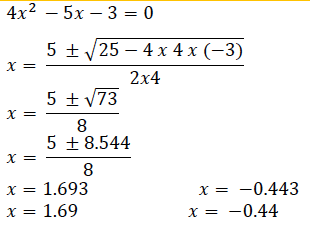(c)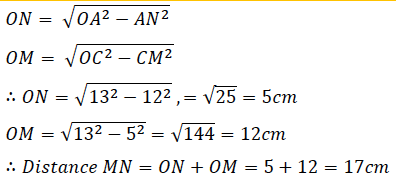### Question 2:

(a) Evaluate without using trigonometric tables,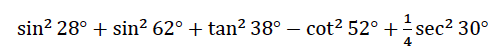(b)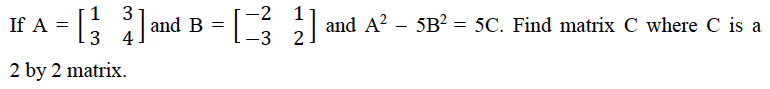(c) Jaya borrowed ₹ 50,000 for 2 years. The rates of interest for two successive years are 12% and 15% respectively. She repays ₹33,000 at the end of the first year. Find the amount she must pay at the end of the second year to clear her debt.

(a)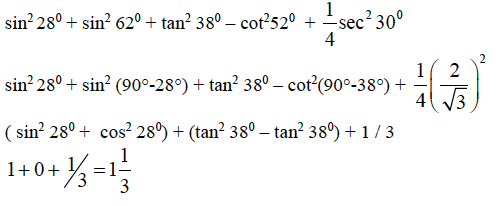(b)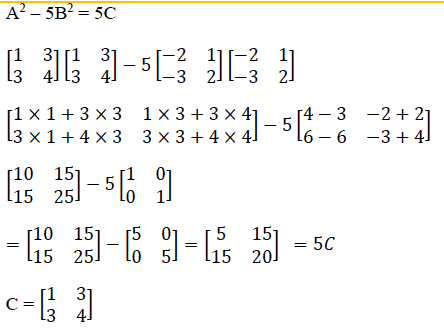(c)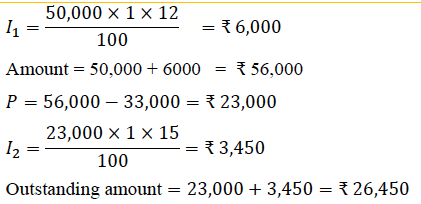### Question 3:

(a) The catalogue price of a computer set is ₹ 42000. The shopkeeper gives a discount of 10% on the listed price. He further gives an off-season discount of 5% on the discounted price. However, sales tax at 8% is charged on the remaining price after the two successive discounts. Find:

(i) the amount of sales tax a customer has to pay

(ii) the total price to be paid by the customer for the computer set.

(b) P(1,–2) is a point on the line segment A(3,–6) and B(x, y) such that AP : PB is equal to 2 : 3. Find the coordinates of B.

(c) The marks of 10 students of a class in an examination arranged in ascending order is as follows:

13, 35, 43, 46, x, x+4, 55, 61, 71, 80

If the median marks is 48, find the value of x. Hence find the mode of the given data.

(a)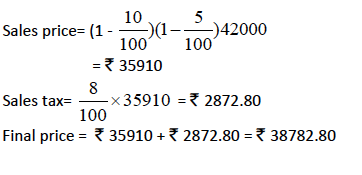(b)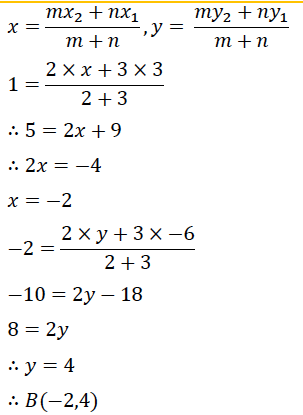(c)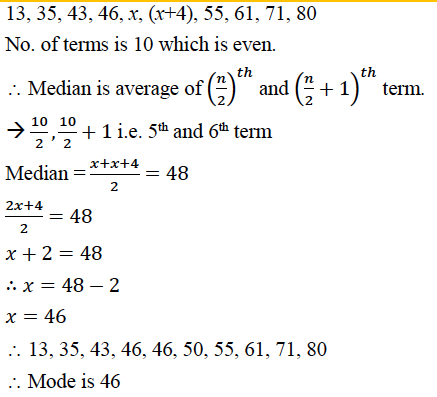### Question 4:

(a) What must be subtracted from 16x3 – 8x2 + 4x + 7 so that the resulting expression has 2x + 1 as a factor?

(b) In the given figure ABCD is a rectangle. It consists of a circle and two semi circles each of which are of radius 5 cm. Find the area of the shaded region. Give your answer correct to three significant figures.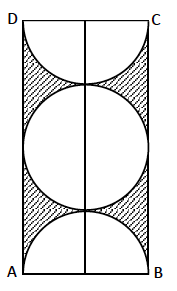(c) Solve the following inequation and represent the solution set on a number line.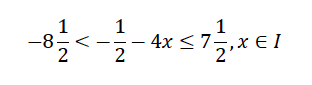(a)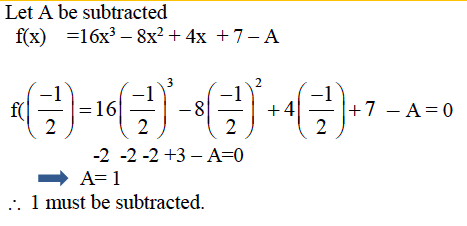(b)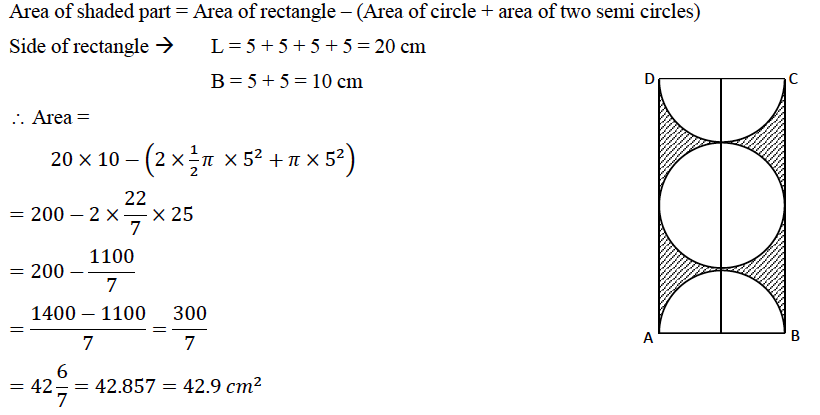(c)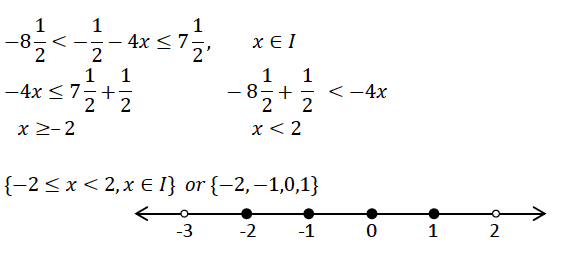### Question 5:

(a)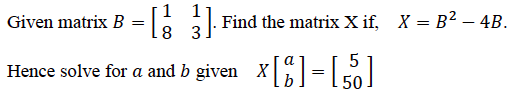(b) How much should a man invest in  50 shares selling at 60 to obtain an income of  450, if the rate of dividend declared is 10%. Also find his yield percent, to the nearest whole number.

(c) Sixteen cards are labelled as a,b,c…….m,n,o,p. They are put in a box and shuffled. A boy is asked to draw a card from the box. What is the probability that the card drawn is:

(i) a vowel.

(ii) a consonant.

(iii) none of the letters of the word median.

(a)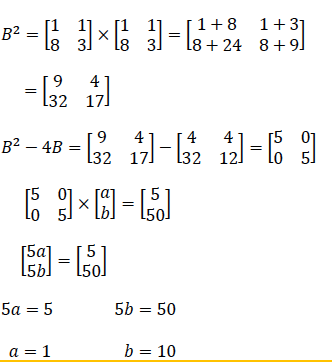(b)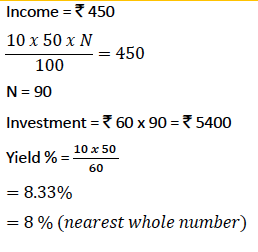(c)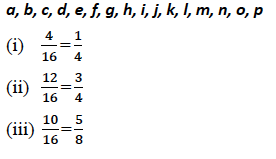### Question 6:

(a) Using a ruler and a compass construct a triangle ABC in which AB = 7cm, ∠CAB=60o and AC = 5cm. Construct the locus of:

(i) points equidistant from AB and AC.

(ii) points equidistant from BA and BC.

Hence construct a circle touching the three sides of the triangle internally.

(b) A conical tent is to accommodate 77 persons. Each person must have 16𝑚3 of air to breathe. Given the radius of the tent as 7𝑚𝑚find the height of the tent and also its curved surface area.

(c)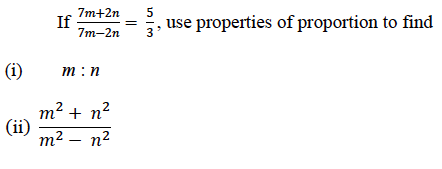(a) Triangle

(i) Bisector of ∠A

(ii) Bisector of ∠B

(iii) Incircle with perpendicular constructed to find radius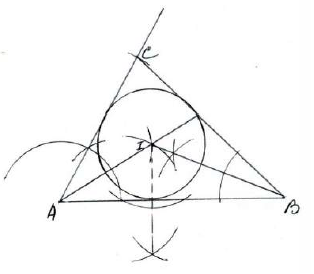(b)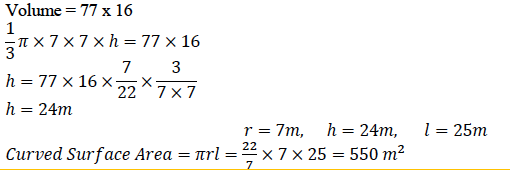(c)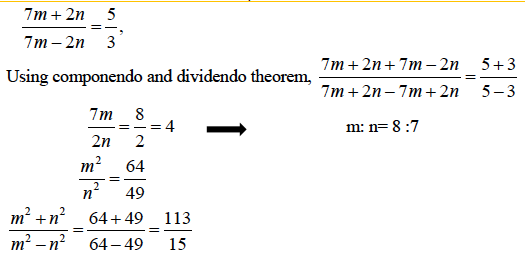### Question 7:

(a) A page from a savings bank account passbook is given below:

 Date Particulars Amount Withdrawn (₹) Amount Deposited (₹) Balance (₹) Jan 7, 2016 B/F 3000.00 Jan 10, 2016 By Cheque 2600.00 5600.0 Feb 8, 2016 To Self 1500.00 4100.00 Apr 6, 2016 By Cheque 2100.00 2000.00 May 4, 2016 By cash 6500.00 8500.00 May 27, 2016 By Cheque 1500.00 10000.00

(i) Calculate the interest for the 6 months from January to June 2016, at 6% per annum.

(ii) If the account is closed on 1st July 2016, find the amount received by the account holder.

(b) Use a graph paper for this question (Take 2 cm = 1 unit on both x and y axis)

(i) Plot the following points:

A(0,4), B(2,3), C(1,1) and D(2,0).

(ii) Reflect points B, C, D on the y-axis and write down their coordinates. Name the images as B’, C’, D’ respectively.

(iii) Join the points A, B, C, D, D’, C’, B’ and A in order, so as to form a closed figure. Write down the equation of the line of symmetry of the figure formed.

(a)

 Qualifying sum for (₹) Jan 5600.00 Feb 4100.00 Mar 4100.00 Apr 2000.00 May 8500.00 June 10000.00 Total 34300.00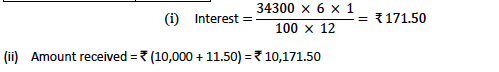(b) Coordinates of images of B, C and D are B’(-2,3), C’(-1,1) and D’(-2,0).

Figure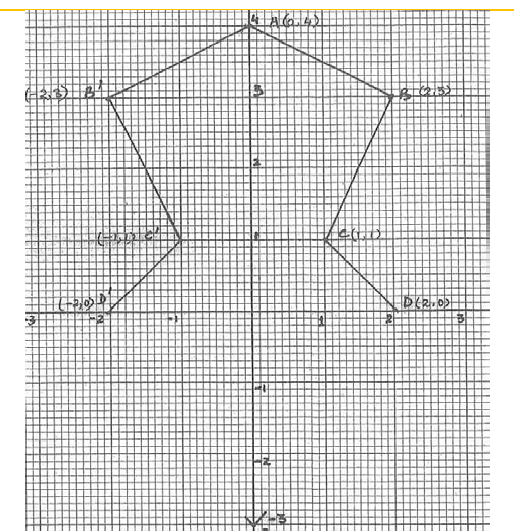Equation of line of symmetry is x = 0

### Question 8:

(a) Calculate the mean of the following distribution using step deviation method.

 Marks 0-10 10-20 20-30 30-40 40-50 50-60 No. of Students 10 9 25 30 16 10

(b)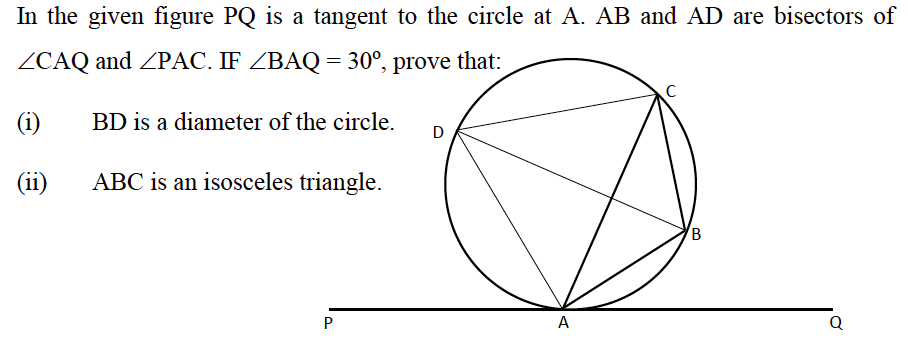(c) The printed price of an air conditioner is ₹ 45,000/-. The wholesaler allows a discount of 10% to the shopkeeper. The shopkeeper sells the article to the customer at a discount of 5% of the marked price. Sales tax (under VAT) is charged at the rate of 12% at every stage. Find:

(i) VAT paid by the shopkeeper to the government.

(ii) The total amount paid by the customer inclusive of tax.

(a)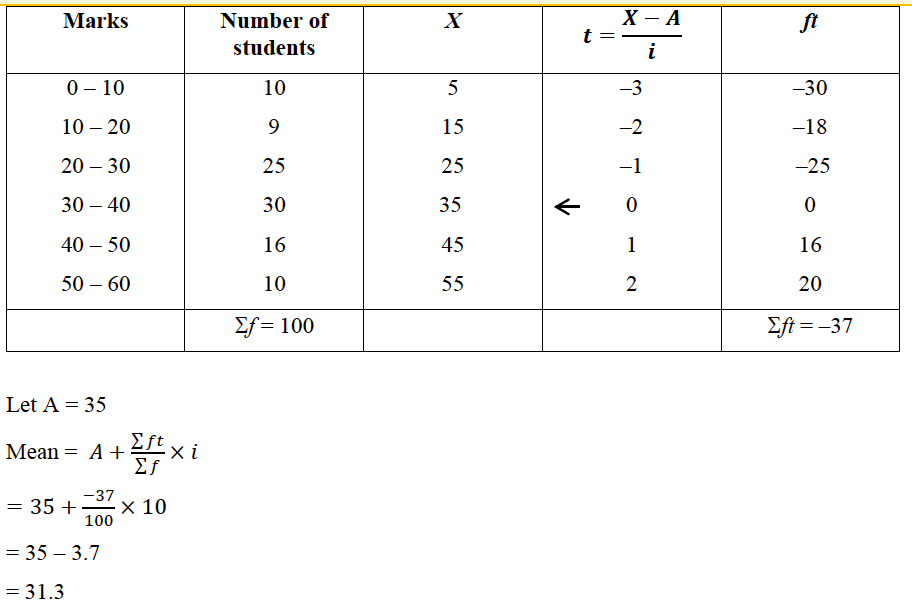(b)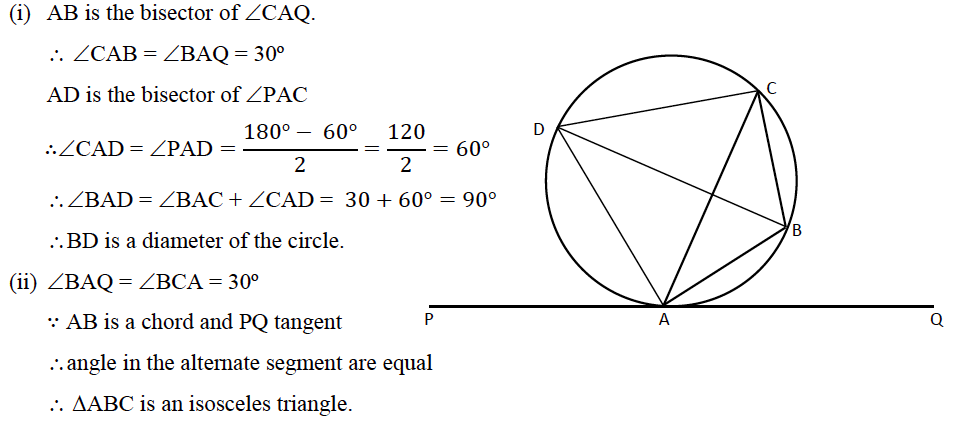(c)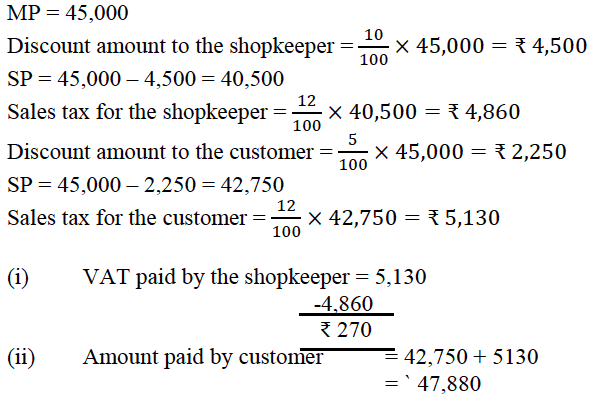### Question 9:

(a) In the figure given, O is the centre of the circle. ∠DAE = 700. Find giving suitable reasons, the measure of:

(i) ∠BCD

(ii) ∠BOD

(iii) ∠OBD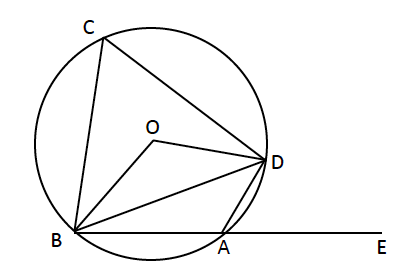(b) A(-1, 3) , B(4, 2) and C(3, -2) are the vertices of a triangle.

(i) Find the coordinates of the centroid G of the triangle.

(ii) Find the equation of the line through G and parallel to AC.

(c) Prove that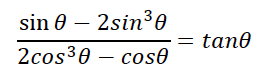(a)∠DAE = ∠BCD (ext. ∠ of a cyclic quad. is equal to interior opp.∠ )

(i) ∠DAE = ∠BCD = 700

(ii) ∠BOD = 2 ∠BCD = 140ᵒ (angle at centre is twice the angle on other segment)

(iii) In ΔOBD, OB = OD (radii of circle)

∴ ∠OBD = ∠ODB = 𝑥ᵒ (say) (at least 2 correct reasons)

𝑥ᵒ + 𝑥ᵒ + 140ᵒ = 180ᵒ (sum of angles of a triangle = 1800)

∴ ∠OBD = 𝑥ᵒ = 20ᵒ

(b)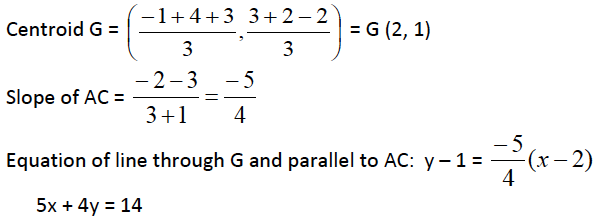(c)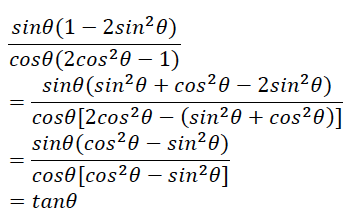### Question 10:

(a) The sum of the ages of Vivek and his younger brother Amit is 47 years. The product of their ages in years is 550. Find their ages.

(b) The daily wages of 80 workers in a project are given below.

 Wages (in ₹) 400-450 450-500 500-550 550-600 600-650 650-700 700-750 No. of Workers 2 6 12 18 24 13 5

Use a graph paper to draw an ogive for the above distribution. (Use a scale of 2 cm = ₹ 50 on x-axis and 2 cm = 10 workers on y-axis). Use your ogive to estimate:

(i) the median wage of the workers.

(ii) the lower quartile wage of workers.

(iii) the number of workers who earn more than ₹ 625 daily.

(a) Let age of Vivek be x years

And of Amit 47 – x years

∴ x (47 – x) = 550

47x – x2 = 550

Or

x2 – 47x + 550 = 0

(x – 25) (x – 22) = 0

∴ x = 25 or age = 22 (not possible)

∴ Vivek’s age is x = 25 years, and Amit’s age is 47 – 25 = 22 years

(b) c.f: 2, 8, 20, 38, 62, 75,80

(For smooth S curve plotted with upper boundries)

(i) Median =Nth/2 term. = 40th term =  605

(ii) Q1 = N/4th term = 20th term =  550

(iii) No. of workers who earn more than  625 = 80-52 = 28(±3)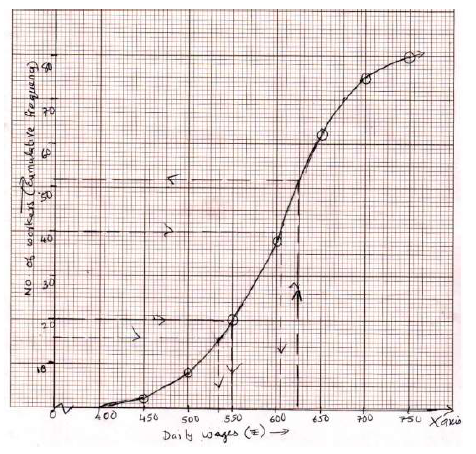### Question 11:

(a) The angles of depression of two ships A and B as observed from the top of a lighthouse 60 m high are 60o and 45o respectively. If the two ships are on the opposite sides of the light house, find the distance between the two ships. Give your answer correct to the nearest whole number.

(b) PQR is a triangle. S is a point on the side QR of ΔPQR such that ∠PSR = ∠QPR.

Given QP = 8 cm, PR = 6 cm and SR = 3 cm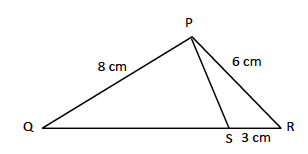(i) Prove ΔPQR ∼ ΔSPR

(ii) Find the length of QR and PS

(iii)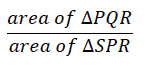(c) Mr. Richard has a recurring deposit account in a bank for 3 years at 7.5% p. a. Simple interest. If he gets ` 8325 as interest at the time of maturity, find:

(i) The monthly deposit

(ii) The maturity value.

(a)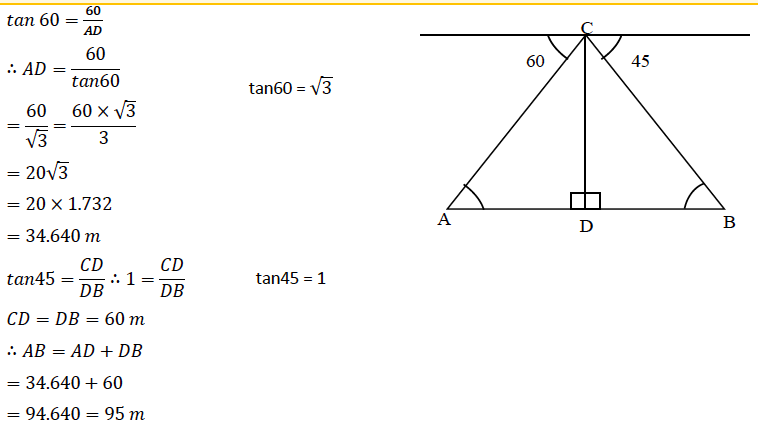(b)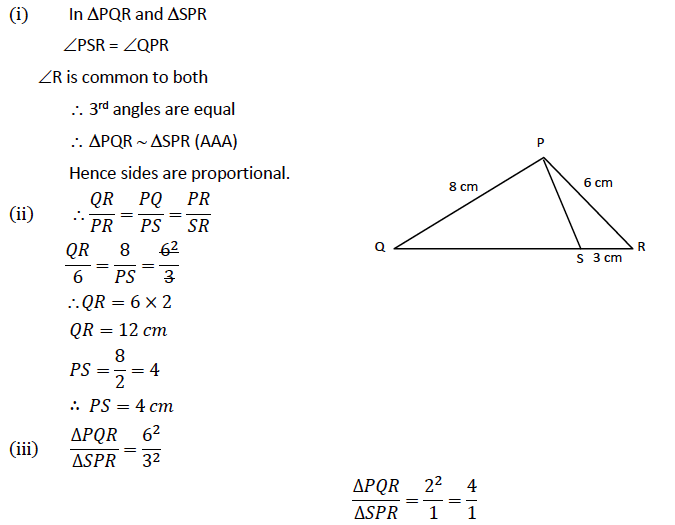(c)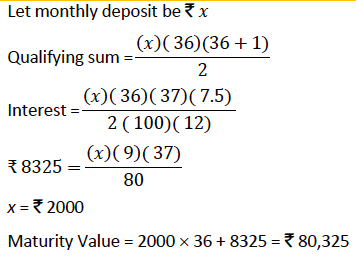We hope ICSE Class 10 Maths Question Paper Solution 2017 must have helped students in their exam preparation. We have also compiled the solutions of other subjects of ICSE Class 10 Previous Years Question Papers at one place. Students can access them by clicking here. Stay tuned to BYJU’S for the latest update on ICSE/CBSE/State Boards/Competitive exams and don’t forget to download the BYJU’S App.